# nLab Feynman propagator

Contents

## Topics in Functional Analysis

#### Riemannian geometry

Riemannian geometry

# Contents

## Idea

What is called the Feynman propagator over a globally hyperbolic spacetime is one of the Green functions for the Klein-Gordon operator $\Box + m^2$ (hence a fundamental solution to the wave equation when the mass $m$ vanishes).

As discussed in detail at S-matrix – Feynman diagrams and renormalization, the Feynman propagator encodes time-ordered products of quantum observables in free field perturbative quantum field theory (in the same way as the Wightman propagator encodes normal ordered products of quantum fields). This implies that the scattering amplitude associated with a Feynman diagram in the Feynman perturbation series expansion of the S-matrix is, away from the locus of coinciding interaction points, a product of Feynman propagators, one for each edge in the Feynman diagram (the extension of distributions of this product of distributions to coinciding points is renormalization).

This is why Feynman propagators are ubiquituous in perturbative quantum field theory: they are the building blocks of perturbative scattering amplitudes.

## Definition

By the discussion at S-matrix – Feynman diagrams and renormalization, the Feynman propagator $\omega_F$ is properly defined to be the linear combination of the chosen Wightman propagator (encoding the vacuum state) and the advanced causal propagator:

###### Definition

(Feynman propagator on globally hyperbolic spacetimes)

Given a time-oriented globally hyperbolic spacetime $\Sigma$ there exists a unique advanced causal propagator $\Delta_A \in \mathcal{D}'(\Sigma \times \Sigma)$ and a Wightman propagator $\omega \in \mathcal{D}'(\Sigma \times \Sigma)$, unique up to addition of a regular distributio (a smooth function). Given a choice of $\omega$ (the vacuum state) then the corresponding Feynman propagator is the sum

$\omega_F \coloneqq \omega + i \Delta_A \,.$

(for Minkowski spacetime this is e.g. Scharf 95 (2.3.41), for general globally hyperbolic spacetimes this is Radzikowski 96, p. 5)

On Minkowski spacetime this may be expressed as a sum of products of distributions of a Heaviside distribution in the time coordinate with the Hadamard distribution and its opposite, and this is often taken as the definition of the Feynman propagator. But the above formula applies to general globally hyperbolic spacetimes.

propagators (i.e. integral kernels of Green functions)
for the wave operator and Klein-Gordon operator
on a globally hyperbolic spacetime such as Minkowski spacetime:

namesymbolwave front setas vacuum exp. value
of field operators
as a product of
field operators
causal propagator\begin{aligned}\Delta_S & = \Delta_+ - \Delta_- \end{aligned}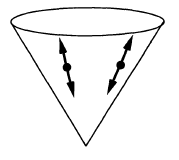$\phantom{A}\,\,\,-$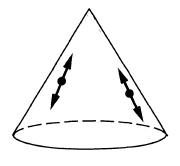\begin{aligned} & i \hbar \, \Delta_S(x,y) = \\ & \left\langle \;\left[\mathbf{\Phi}(x),\mathbf{\Phi}(y)\right]\; \right\rangle \end{aligned}Peierls-Poisson bracket
advanced propagator$\Delta_+$\begin{aligned} & i \hbar \, \Delta_+(x,y) = \\ & \left\{ \array{ \left\langle \; \left[ \mathbf{\Phi}(x),\mathbf{\Phi}(y) \right] \; \right\rangle &\vert& x \geq y \\ 0 &\vert& y \geq x } \right. \end{aligned}future part of
Peierls-Poisson bracket
retarded propagator$\Delta_-$\begin{aligned} & i \hbar \, \Delta_-(x,y) = \\ & \left\{ \array{ \left\langle \; \left[\mathbf{\Phi}(x),\mathbf{\Phi}(y) \right] \; \right\rangle &\vert& y \geq x \\ 0 &\vert& x \geq y } \right. \end{aligned}past part of
Peierls-Poisson bracket
Wightman propagator\begin{aligned} \Delta_H &= \tfrac{i}{2}\left( \Delta_+ - \Delta_-\right) + H\\ & = \tfrac{i}{2}\Delta_S + H \\ & = \Delta_F - i \Delta_- \end{aligned}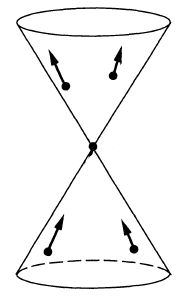\begin{aligned} & \hbar \, \Delta_H(x,y) \\ & = \left\langle \; \mathbf{\Phi}(x) \mathbf{\Phi}(y) \; \right\rangle \\ & = \underset{ = 0 }{\underbrace{\left\langle \; : \mathbf{\Phi}(x) \mathbf{\Phi}(y) : \; \right\rangle}} \\ & \phantom{=} + \left\langle \; \left[ \mathbf{\Phi}^{(-)}(x), \mathbf{\Phi}^{(+)}(y) \right] \; \right\rangle \end{aligned}positive frequency of
Peierls-Poisson bracket,
Wick algebra-product,
2-point function
$\phantom{=}$ of vacuum state
$\phantom{=}$ or generally of
$\phantom{=}$ Hadamard state
Feynman propagator\begin{aligned}\Delta_F & = \tfrac{i}{2}\left( \Delta_+ + \Delta_- \right) + H \\ & = i \Delta_D + H \\ & = \Delta_H + i \Delta_- \end{aligned}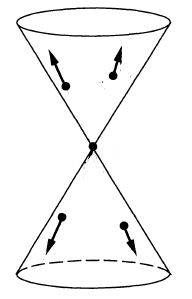\begin{aligned} & \hbar \, \Delta_F(x,y) \\ & = \left\langle \; T\left( \; \mathbf{\Phi}(x)\mathbf{\Phi}(y) \;\right) \; \right\rangle \\ & = \left\{ \array{ \left\langle \; \mathbf{\Phi}(x)\mathbf{\Phi}(x) \; \right\rangle &\vert& x \geq y \\ \left\langle \; \mathbf{\Phi}(y) \mathbf{\Phi}(x) \; \right\rangle &\vert& y \geq x } \right.\end{aligned}time-ordered product

## Examples

### For Klein-Gordon operator on Minkowski spacetime

On Minkowski spacetime $\mathbb{R}^{p,1}$ consider the Klein-Gordon operator

$\eta^{\mu \nu} \frac{\partial}{\partial x^\mu} \frac{\partial}{\partial x^\nu} \Phi - \left( \tfrac{m c}{\hbar} \right)^2 \Phi \;=\; 0 \,.$

Its Fourier transform is

$- k_\mu k^\mu - \left( \tfrac{m c}{\hbar} \right)^2 \;=\; (k_0)^2 - {\vert \vec k\vert}^2 - \left( \tfrac{m c}{\hbar} \right)^2 \,.$

The dispersion relation of this equation we write

(1)$\omega(\vec k) \;\coloneqq\; + c \sqrt{ {\vert \vec k \vert}^2 + \left( \tfrac{m c}{\hbar}\right)^2 } \,,$

where on the right we choose the non-negative square root.

$\,$

We now discuss

$\,$

advanced and retarded propagators for Klein-Gordon equation on Minkowski spacetime

###### Proposition

(mode expansion of advanced and retarded propagators for Klein-Gordon operator on Minkowski spacetime)

The advanced and retarded Green functions $G_\pm$ of the Klein-Gordon operator on Minkowski spacetime are given by integral kernels (“propagators”)

$\Delta_\pm \in \mathcal{D}'(\mathbb{R}^{p,1}\times \mathbb{R}^{p,1})$

by (in generalized function-notation)

$G_\pm(\Phi) \;=\; \underset{\mathbb{R}^{p,1}}{\int} \Delta_{\pm}(x,y) \Phi(y) \, dvol(y)$

where the advanced and retarded propagators $\Delta_{\pm}(x,y)$ have the following equivalent expressions:

(2)\begin{aligned} \Delta_\pm(x-y) & = \frac{1}{(2\pi)^{p+1}} \underset{ {\epsilon \in (0,\infty)} \atop {\epsilon \to 0} }{\lim} \int \int \frac{ e^{i k_0 (x^0 - y^0)} e^{i \vec k \cdot (\vec x - \vec y)} }{ (k_0 \mp i\epsilon)^2 - {\vert \vec k\vert}^2 -\left( \tfrac{m c}{\hbar}\right)^2 } \, d k_0 \, d^p \vec k \\ & = \left\{ \array{ \frac{\pm i}{(2\pi)^{p}} \int \frac{1}{2\omega(\vec k)/c} \left( e^{+i \omega(\vec k)(x^0 - y^0)/c + i \vec k \cdot (\vec x -\vec y)} - e^{-i \omega(\vec k)(x^0 - y^0)/c + i \vec k \cdot (\vec x - \vec y) } \right) d^p \vec k & \vert & \text{if} \, \pm (x^0 - y^0) \gt 0 \\ 0 & \vert & \text{otherwise} } \right. \\ & = \left\{ \array{ \frac{\mp 1}{(2\pi)^{p}} \int \frac{1}{\omega(\vec k)/c} \sin\left( \omega(\vec k)(x^0 - y^0)/c \right) e^{i \vec k \cdot (\vec x - \vec y) } d^p \vec k & \vert & \text{if} \, \pm (x^0 - y^0) \gt 0 \\ 0 & \vert & \text{otherwise} } \right. \end{aligned}

Here $\omega(\vec k)$ denotes the dispersion relation (1) of the Klein-Gordon equation.

###### Proof

The Klein-Gordon operator is a Green hyperbolic differential operator (this example) therefore its advanced and retarded Green functions exist uniquely (this prop.). Moreover, this prop. says that they are continuous linear functionals with respect to the topological vector space structures on spaces of smooth sections (this def.). In the case of the Klein-Gordon operator this just means that

$G_{\pm} \;\colon\; C^\infty_{cp}(\mathbb{R}^{p,1}) \longrightarrow C^\infty_{\pm cp}(\mathbb{R}^{p,1})$

are continuous linear functionals in the standard sense of distributions. Therefore the Schwartz kernel theorem implies the existence of integral kernels being distributions in two variables

$\Delta_{\pm} \in \mathcal{D}(\mathbb{R}^{p,1} \times \mathbb{R}^{p,1})$

such that, in the notation of generalized functions,

$(G_\pm \alpha)(x) \;=\; \underset{\mathbb{R}^{p,1}}{\int} \Delta_{\pm}(x,y) \alpha(y) \, dvol(y) \,.$

These integral kernels are the advanced/retarded “propagators”. We now compute these integral kernels by making an Ansatz and showing that it has the defining properties, which identifies them by the uniqueness statement of this prop..

We make use of the fact that the Klein-Gordon equation is invariant under the defnining action of the Poincaré group on Minkowski spacetime, which is a semidirect product group of the translation group and the Lorentz group.

Since the Klein-Gordon operator is invariant, in particular, under translations in $\mathbb{R}^{p,1}$ it is clear that the propagators, as a distribution in two variables, depend only on the difference of its two arguments

$\Delta_{\pm}(x,y) = \Delta_{\pm}(x-y) \,.$

Since moreover the Klein-Gordon operator is formally self-adjoint (this prop.) this implies that for $P$ the Klein the equation (?)

$P \circ G_\pm = id$

is equivalent to the equation (?)

$G_\pm \circ P = id \,.$

Therefore it is sufficient to solve for the first of these two equation, subject to the defining support conditions. In terms of the propagator integral kernels this means that we have to solve the distributional equation

(3)$\left( \eta^{\mu \nu} \frac{\partial}{\partial x^\mu} \frac{\partial}{\partial x^\nu} - \left( \tfrac{m c}{\hbar} \right)^2 \right) \Delta_\pm(x-y) \;=\; \delta(x-y)$

subject to the condition that the distributional support is

$supp\left( \Delta_{\pm}(x-y) \right) \subset \left\{ {\vert x-y\vert^2_\eta}\lt 0 \;\,,\; \pm(x^0 - y^ 0) \gt 0 \right\} \,.$

We make the Ansatz that we assume that $\Delta_{\pm}$, as a distribution in a single variable $x-y$, is a tempered distribution

$\Delta_\pm \in \mathcal{S}'(\mathbb{R}^{p,1}) \,,$

hence amenable to Fourier transform of distributions. If we do find a solution this way, it is guaranteed to be the unique solution by this prop..

By this prop. the distributional Fourier transform of equation (3) is

(4)\begin{aligned} \left( - \eta^{\mu \nu} k_\mu k_\nu - \left( \tfrac{m c}{\hbar} \right)^2 \right) \widehat{\Delta_{\pm}}(k) & = \widehat{\delta}(k) \\ & = 1 \end{aligned} \,,

where in the second line we used the Fourier transform of the delta distribution from this example.

Notice that this implies that the Fourier transform of the causal propagator

$\Delta_S \coloneqq \Delta_+ - \Delta_-$

satisfies the homogeneous equation:

(5)$\left( - \eta^{\mu \nu} k_\mu k_\nu - \left( \tfrac{m c}{\hbar} \right)^2 \right) \widehat{\Delta_S}(k) \;=\; 0 \,,$

Hence we are now reduced to finding solutions $\widehat{\Delta_\pm} \in \mathcal{S}'(\mathbb{R}^{p,1})$ to (4) such that their Fourier inverse $\Delta_\pm$ has the required support properties.

We discuss this by a variant of the Cauchy principal value:

Suppose the following limit of non-singular distributions in the variable $k \in \mathbb{R}^{p,1}$ exists in the space of distributions

(6)$\underset{ {\epsilon \in (0,\infty)} \atop { \epsilon \to 0 } }{\lim} \frac{1}{ (k_0 \mp i \epsilon)^2 - {\vert \vec k\vert^2} - \left( \tfrac{m c}{\hbar} \right)^2 } \;\in\; \mathcal{D}'(\mathbb{R}^{p,1})$

meaning that for each bump function $b \in C^\infty_{cp}(\mathbb{R}^{p,1})$ the limit in $\mathbb{C}$

$\underset{ {\epsilon \in (0,\infty)} \atop { \epsilon \to 0 } }{\lim} \underset{\mathbb{R}^{p,1}}{\int} \frac{b(k)}{ (k_0\mp i \epsilon)^2 - {\vert \vec k\vert}^2 - \left( \tfrac{m c}{\hbar} \right)^2 } d^{p+1}k \;\in\; \mathbb{C}$

exists. Then this limit is clearly a solution to the distributional equation (4) because on those bump functions $b(k)$ which happen to be products with $\left(-\eta^{\mu \nu}k_\mu k-\nu - \left( \tfrac{m c}{\hbar}\right)^2\right)$ we clearly have

\begin{aligned} \underset{ {\epsilon \in (0,\infty)} \atop { \epsilon \to 0 } }{\lim} \underset{\mathbb{R}^{p,1}}{\int} \frac{ \left( -\eta^{\mu \nu} k_\mu k_\nu - \left( \tfrac{m c}{\hbar} \right)^2 \right) b(k) }{ (k_0\mp i \epsilon)^2 - {\vert \vec k\vert}^2 - \left( \tfrac{m c}{\hbar} \right)^2 } d^{p+1}k & = \underset{\mathbb{R}^{p,1}}{\int} \underset{= 1}{ \underbrace{ \underset{ {\epsilon \in (0,\infty)} \atop { \epsilon \to 0 } }{\lim} \frac{ \left( -\eta^{\mu \nu} k_\mu k_\nu - \left( \tfrac{m c}{\hbar} \right)^2 \right) }{ (k_0\mp i \epsilon)^2 - {\vert \vec k\vert}^2 - \left( \tfrac{m c}{\hbar} \right)^2 } } } b(k)\, d^{p+1}k \\ & = \langle 1, b\rangle \,. \end{aligned}

Moreover, if the limiting distribution (6) exists, then it is clearly a tempered distribution, hence we may apply Fourier inversion to obtain Green functions

(7)$\Delta_{\pm}(x,y) \;\coloneqq\; \underset{ {\epsilon \in (0,\infty)} \atop {\epsilon \to 0} }{\lim} \frac{1}{(2\pi)^{p+1}} \underset{\mathbb{R}^{p,1}}{\int} \frac{e^{i k_\mu (x-y)^\mu}}{ (k_0 \mp i \epsilon )^2 - {\vert \vec k\vert}^2 - \left(\tfrac{m c}{\hbar}\right)^2 } d k_0 d^p \vec k \,.$

To see that this is the correct answer, we need to check the defining support property.

Finally, by the Fourier inversion theorem, to show that the limit (6) indeed exists it is sufficient to show that the limit in (7) exists.

We compute as follows

(8)\begin{aligned} \Delta_\pm(x-y) & = \frac{1}{(2\pi)^{p+1}} \underset{ {\epsilon \in (0,\infty)} \atop {\epsilon \to 0} }{\lim} \int \int \frac{ e^{i k_0 (x^0 - y^0)} e^{i \vec k \cdot (\vec x - \vec y)} }{ (k_0 \mp i\epsilon)^2 - {\vert \vec k\vert}^2 -\left( \tfrac{m c}{\hbar}\right)^2 } \, d k_0 \, d^p \vec k \\ & = \frac{1}{(2\pi)^{p+1}} \underset{ {\epsilon \in (0,\infty)} \atop {\epsilon \to 0} }{\lim} \int \int \frac{ e^{i k_0 (x^0 - y^0)} e^{i \vec k \cdot (\vec x - \vec y)} }{ (k_0 \mp i \epsilon)^2 - \left(\omega(\vec k)/c\right)^2 } \, d k_0 \, d^p \vec k \\ &= \frac{1}{(2\pi)^{p+1}} \underset{ {\epsilon \in (0,\infty)} \atop {\epsilon \to 0} }{\lim} \int \int \frac{ e^{i k_0 (x^0 - y^0)} e^{i \vec k \cdot (\vec x - \vec y)} }{ \left( (k_0 \mp i\epsilon) - \omega(\vec k)/c \right) \left( (k_0 \mp i \epsilon) + \omega(\vec k)/c \right) } \, d k_0 \, d^p \vec k \\ & = \left\{ \array{ \frac{\pm i}{(2\pi)^{p}} \int \frac{1}{2\omega(\vec k)/c} \left( e^{i \omega(\vec k)(x^0 - y^0)/c + i \vec k \cdot (\vec x -\vec y)} - e^{-i \omega(\vec k)(x^0 - y^0)/c + i \vec k \cdot (\vec x - \vec y)} \right) d^p \vec k & \vert & \text{if} \, \pm (x^0 - y^0) \gt 0 \\ 0 & \vert & \text{otherwise} } \right. \\ & = \left\{ \array{ \frac{\mp 1}{(2\pi)^{p}} \int \frac{1}{\omega(\vec k)/c} \sin\left( \omega(\vec k)(x^0 - y^0)/c \right) e^{i \vec k \cdot (\vec x - \vec y) } d^p \vec k & \vert & \text{if} \, \pm (x^0 - y^0) \gt 0 \\ 0 & \vert & \text{otherwise} } \right. \end{aligned}

where $\omega(\vec k)$ denotes the dispersion relation (1) of the Klein-Gordon equation. The last step is simply the application of Euler's formula $\sin(\alpha) = \tfrac{1}{2 i }\left( e^{i \alpha} - e^{- i \alpha}\right)$.

Here the key step is the application of Cauchy's integral formula in the fourth step. We spell this out now for $\Delta_+$, the discussion for $\Delta_-$ is the same, just with the appropriate signs reversed.

1. If $(x^0 - y^0) \gt 0$ thn the expression $e^{ik_0 (x^0 - y^0)}$ decays with positive imaginary part of $k_0$, so that we may expand the integration domain into the upper half plane as
\begin{aligned} \int_{-\infty}^\infty d k_0 & = \phantom{+} \int_{-\infty}^0 d k_0 + \int_{0}^{+ i \infty} d k_0 \\ & = + \int_{+i \infty}^0 d k_0 + \int_0^\infty d k_0 \,; \end{aligned}

Conversely, if $(x^0 - y^0) \lt 0$ then we may analogously expand into the lower half plane.

1. This integration domain may then further be completed to two contour integrations. For the expansion into the upper half plane these encircle counter-clockwise the poles at $\pm \omega(\vec k)+ i\epsilon \in \mathbb{C}$, while for expansion into the lower half plane no poles are being encircled.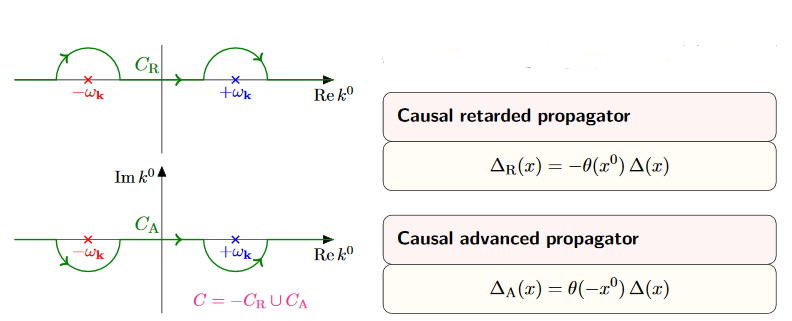1. Apply Cauchy's integral formula to find in the case $(x^0 - y^0)\gt 0$ the sum of the residues at these two poles times $2\pi i$, zero in the other case. (For the retarded propagator we get $- 2 \pi i$ times the residues, because now the contours encircling non-trivial poles go clockwise).

2. The result is now non-singular at $\epsion = 0$ and therefore the limit $\epsilon \to 0$ is now computed by evaluating at $\epsilon = 0$.

This computation shows a) that the limiting distribution indeed exists, and b) that the support of $\Delta_+$ is in the future, and that of $\Delta_-$ is in the past.

Hence it only remains to see now that the support of $\Delta_\pm$ is inside the causal cone. But this follows from the previous argument, by using that the Klein-Gordon equation is invariant under Lorentz transformations: This implies that the support is in fact in the future of every spacelike slice through the origin in $\mathbb{R}^{p,1}$, hence in the closed future cone of the origin.

###### Corollary

(causal propagator is skew-symmetric)

Under reversal of arguments the advanced and retarded causal propagators are related by

(9)$\Delta_{\pm}(y-x) = \Delta_\mp(x-y) \,.$

It follows that the causal propagator $\Delta \coloneqq \Delta_+ - \Delta_-$ is skew-symmetric in its arguments:

$\Delta_S(x-y) = - \Delta_S(y-x) \,.$
###### Proof

By prop. we have with (2)

\begin{aligned} \Delta_\pm(y-x) & = \left\{ \array{ \frac{\pm i}{(2\pi)^{p}} \int \frac{1}{2\omega(\vec k)/c} \left( e^{-i \omega(\vec k)(x^0 - y^0)/c - i \vec k \cdot (\vec x -\vec y)} - e^{+i \omega(\vec k)(x^0 - y^0)/c - i \vec k \cdot (\vec x - \vec y) } \right) d^p \vec k & \vert & \text{if} \, \mp (x^0 - y^0) \gt 0 \\ 0 & \vert & \text{otherwise} } \right. \\ & = \left\{ \array{ \frac{\pm i}{(2\pi)^{p}} \int \frac{1}{2\omega(\vec k)/c} \left( e^{-i \omega(\vec k)(x^0 - y^0)/c + i \vec k \cdot (\vec x -\vec y)} - e^{+i \omega(\vec k)(x^0 - y^0)/c - i \vec k \cdot (\vec x - \vec y) } \right) d^p \vec k & \vert & \text{if} \, \mp (x^0 - y^0) \gt 0 \\ 0 & \vert & \text{otherwise} } \right. \\ & = \left\{ \array{ \frac{\mp i}{(2\pi)^{p}} \int \frac{1}{2\omega(\vec k)/c} \left( e^{+i \omega(\vec k)(x^0 - y^0)/c + i \vec k \cdot (\vec x -\vec y)} - e^{-i \omega(\vec k)(x^0 - y^0)/c - i \vec k \cdot (\vec x - \vec y) } \right) d^p \vec k & \vert & \text{if} \, \mp (x^0 - y^0) \gt 0 \\ 0 & \vert & \text{otherwise} } \right. \\ & = \Delta_\mp(x-y) \end{aligned}

Here in the second step we applied change of integration variables $\vec k \mapsto - \vec k$ (which introduces no sign because in addition to $d \vec k \mapsto - d \vec k$ the integration domain reverses orientation).

$\,$

causal propagator

###### Proposition

(mode expansion of causal propagator for Klein-Gordon equation on Minkowski spacetime)

The causal propagator (?) for the Klein-Gordon equation for mass $m$ on Minkowski spacetime $\mathbb{R}^{p,1}$ is given, in generalized function notation, by

(10)\begin{aligned} \Delta_S(x,y) & = \frac{+ i}{(2\pi)^{p}} \int \frac{1}{2\omega(\vec k)/c} \left( e^{i \omega(\vec k)(x^0 - y^0)/c + i \vec k \cdot (\vec x -\vec y)} - e^{-i \omega(\vec k)(x^0 - y^0)/c + i \vec k \cdot (\vec x - \vec y)} \right) d^p \vec k \\ & = \frac{-1}{(2\pi)^p} \int \frac{1}{\omega(\vec k)/c} \sin\left( \omega(\vec k)(x^0 - y^0)/c \right) e^{i \vec k \cdot (\vec x -\vec y)} d^p \vec k \,, \end{aligned}

where in the second line we used Euler's formula $sin(\alpha)= \tfrac{1}{2i}\left( e^{i \alpha} - e^{-i \alpha} \right)$.

In particular this shows that the causal propagator is real, in that it is equal to its complex conjugate

(11)$\left(\Delta_S(x,y)\right)^\ast = \Delta_S(x,y) \,.$
###### Proof

By definition and using the expression from prop. for the advanced and retarded causal propagators we have

\begin{aligned} \Delta_S(x,y) & \coloneqq \Delta_+(x,y) - \Delta_-(x,y) \\ & = \left\{ \array{ \frac{+ i}{(2\pi)^{p}} \int \frac{1}{2\omega(\vec k)/c} \left( e^{i \omega(\vec k)(x^0 - y^0)/c + i \vec k \cdot (\vec x -\vec y)} - e^{-i \omega(\vec k)(x^0 - y^0)/c + i \vec k \cdot (\vec x - \vec y)} \right) d^p \vec k & \vert & \text{if} \, + (x^0 - y^0) \gt 0 \\ \frac{(-1) (-1) i}{(2\pi)^{p}} \int \frac{1}{2\omega(\vec k)/c} \left( e^{i \omega(\vec k)(x^0 - y^0)/c + i \vec k \cdot (\vec x -\vec y)} - e^{-i \omega(\vec k)(x^0 - y^0)/c + i \vec k \cdot (\vec x - \vec y)} \right) d^p \vec k & \vert & \text{if} \, - (x^0 - y^0) \gt 0 } \right. \\ & = \frac{+ i}{(2\pi)^{p}} \int \frac{1}{2\omega(\vec k)/c} \left( e^{i \omega(\vec k)(x^0 - y^0)/c + i \vec k \cdot (\vec x -\vec y)} - e^{-i \omega(\vec k)(x^0 - y^0)/c + i \vec k \cdot (\vec x - \vec y)} \right) d^p \vec k \\ & = \frac{-1}{(2\pi)^p} \int \frac{1}{\omega(\vec k)/c} \sin\left( \omega(\vec k)(x^0 - y^0)/c \right) e^{i \vec k \cdot (\vec x -\vec y)} d^p \vec k \end{aligned}

For the reality, notice from the last line that

\begin{aligned} \left(\Delta_S(x,y)\right)^\ast & = \frac{-1}{(2\pi)^p} \int \frac{1}{\omega(\vec k)/c} \sin\left( \omega(\vec k)(x^0 - y^0)/c \right) e^{-i \vec k \cdot (\vec x -\vec y)} d^p \vec k \\ & = \frac{-1}{(2\pi)^p} \int \frac{1}{\omega(\vec k)/c} \sin\left( \omega(\vec k)(x^0 - y^0)/c \right) e^{+i \vec k \cdot (\vec x -\vec y)} d^p \vec k \\ & = \Delta_S(x,y) \,, \end{aligned}

where in the last step we used the change of integration variables $\vec k \mapsto - \vec k$ (whih introduces no sign, since on top of $d \vec k \mapsto - d \vec k$ the orientation of the integration domain changes).

We consider a couple of equivalent expressions for the causal propagator:

###### Proposition

(causal propagator for Klein-Gordon operator on Minkowski spacetime as a contour integral)

The causal propagator for the Klein-Gordon equation at mass $m$ on Minkowski spacetime has the following equivalent expression, as a generalized function, given as a contour integral along a curve $C(\vec k)$ going counter-clockwise around the two poles at $k_0 = \pm \omega(\vec k)/c$:

$\Delta_S(x,y) \;=\; (2\pi)^{-(p+1)} \int \underset{C(\vec k)}{\oint} \frac{e^{i k_\mu (x-y)^\mu}}{ -k_\mu k^\mu - \left( \tfrac{m c}{\hbar} \right)^2g } \,d k_0 \,d^{p} k \,.$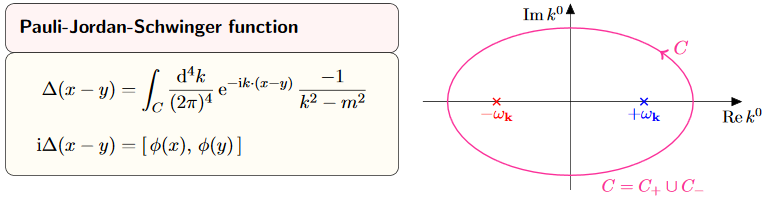graphics grabbed from Kocic 16

###### Proof

By Cauchy's integral formula we compute as follows:

\begin{aligned} (2\pi)^{-(p+1)} \int \underset{C(\vec k)}{\oint} \frac{e^{i k_\mu (x^\mu - y^\mu)}}{ -k_\mu k^\mu - \left( \tfrac{m c}{\hbar} \right)^2 } \,d k_0 \,d^{p} k & = (2\pi)^{-(p+1)} \int \underset{C(\vec k)}{\oint} \frac{ e^{i k_0 x^0} e^{ i \vec k \cdot (\vec x - \vec y)} }{ k_0^2 - \omega(\vec k)^2/c^2 } \,d k_0 \,d^p \vec k \\ & = (2\pi)^{-(p+1)} \int \underset{C(\vec k)}{\oint} \frac{ e^{i k_0 (x^0 - y^0)} e^{i \vec k \cdot (\vec x - \vec y)} }{ ( k_0 + \omega(\vec k)/c ) ( k_0 - \omega(\vec k)/c ) } \,d k_0 \,d^p \vec k \\ & = (2\pi)^{-(p+1)} 2\pi i \int \left( \frac{ e^{i \omega(\vec k) (x^0 - y^0)/c} e^{i \vec k \cdot (\vec x - \vec y)} } { 2 \omega(\vec k)/c } - \frac{ e^{ - i \omega(\vec k) (x^0 - y^0)/c} e^{i \vec k \cdot (\vec x - \vec y)} }{ 2 \omega(\vec k)/c } \right) \,d^p \vec k \\ & = i (2\pi)^{-p} \int \frac{1}{\omega(\vec k)/c} sin\left( \omega(\vec k)(x^0 - y^0)/c \right) e^{i \vec k \cdot (\vec x - \vec y)} \,d^p \vec k \,. \end{aligned}

The last line is the expression for the causal propagator from prop.

###### Proposition

(causal propagator as Fourier transform of delta distribution on the Fourier transformed Klein-Gordon operator)

The causal propagator for the Klein-Gordon equation at mass $m$ on Minkowski spacetime has the following equivalent expression, as a generalized function:

$\Delta_S(x,y) \;=\; i (2\pi)^{-p} \int \delta\left( k_\mu k^\mu + \left( \tfrac{m c}{\hbar}\right)^2 \right) sgn( k_0 ) e^{ i k_\mu (x-y)^\mu } d^{p+1} k \,,$

where the integrand is the product of the sign function of $k_0$ with the delta distribution of the Fourier transform of the Klein-Gordon operator and a plane wave factor.

###### Proof

By decomposing the integral over $k_0$ into its negative and its positive half, and applying the change of integration variables $k_0 = \pm\sqrt{h}$ we get

\begin{aligned} i (2\pi)^{-p} \int \delta\left( k_\mu k^\mu + \left( \tfrac{m c}{\hbar}\right)^2 \right) sgn( k_0 ) e^{ i k_\mu (x-y)^\mu } d^{p+1} k & = + i (2\pi)^{-p} \int \int_0^\infty \delta\left( -k_0^2 + \vec k^2 + \left( \tfrac{m c}{\hbar}\right)^2 \right) e^{ i k_0 (x^0 - y^0) + i \vec k \cdot (\vec x - \vec y)} d k_0 \, d^p \vec k \\ & \phantom{=} - i (2\pi)^{-p} \int \int_{-\infty}^0 \delta\left( -k_0^2 + \vec k^2 + \left(\tfrac{m c}{\hbar}\right)^2 \right) e^{ i k_0 (x^0 - y^0)+ i \vec k \cdot (\vec x - \vec y) } d k_0 \, d^{p} \vec k \\ & = +i (2\pi)^{-p} \int \int_0^\infty \frac{1}{2 \sqrt{h}} \delta\left( -h + \omega(\vec k)^2/c^2 \right) e^{ + i \sqrt{h} (x^0 - y^0) + i \vec k \cdot \vec x } d h \, d^{p} \vec k \\ & \phantom{=} - i (2\pi)^{-p} \int \int_0^\infty \frac{1}{2 \sqrt{h}} \delta\left( - h + \omega(\vec k)^2/c^2 \right) e^{ - i \sqrt{h} (x^0 - y^0) + i \vec k \cdot \vec x } d h \, d^{p} \vec k \\ & = +i (2\pi)^{-p} \int \frac{1}{2 \omega(\vec k)/c} e^{ i \omega(\vec k) (x-y)^0/c + i \vec k \cdot \vec x} d^{p} \vec k \\ & \phantom{=} - i (2\pi)^{-p} \int \frac{1}{2 \omega(\vec k)/c} e^{ - i \omega(\vec k) (x-y)^0/c + i \vec k \cdot \vec x } d^{p} \vec k \\ & = -(2 \pi)^{-p} \int \frac{1}{\omega(\vec k)/c} sin\left( \omega(\vec k)(x-y)^0/c \right) e^{i \vec k \cdot (\vec x - \vec y)} \end{aligned}

The last line is the expression for the causal propagator from prop. .

###### Proposition

(singular support of the causal propagator of the Klein-Gordon equation on Minkowski spacetime is the light cone)

The singular support of the causal propagator for the Klein-Gordon equation on Minkowski spacetime is the light cone of the origin:

$\Delta_S(x,y) \;\propto\; sgn(x^0 - y^0) \delta\left( -{\vert x-y\vert}^2_\eta \right) - \Theta\left( -{\vert x-y\vert}^2_\eta \right) \left( \text{non-singular} \right) \,.$

(e.g. Scharf 95 (2.3.18))

###### Proof

Consider the formula for the causal propagator in terms of the mode expansion (10). Since the integrand here depends on the wave vector $\vec k$ only via its norm ${\vert \vec k\vert}$ and the angle $\theta$ it makes with the given spacetime vector via

$\vec k \cdot (\vec x - \vec y) \;=\; {\vert \vec k\vert} \, {\vert \vec x\vert} \, \cos(\theta)$

we may express the integration in terms of polar coordinates as follws:

\begin{aligned} \Delta_S(x - y) & = \frac{-1}{(2\pi)^p} \int \frac{1}{2 \omega(\vec k)/c} \sin\left( \omega(\vec k)(x^0 - y^0)/c \right) e^{i \vec k \cdot (\vec x - \vec y)} \, d^p \vec k \\ & = \frac{- vol_{S^{p-2}}}{(2\pi)^p} \underset{{\vert \vec k\vert} \in \mathbb{R}_{\geq 0}}{\int} \underset{ \theta \in [0,\pi] }{\int} \frac{ 1 }{ \omega(\vec k)/c } \sin\left( \omega(\vec k) (x^0 - y^0) /c \right) e^{ i {\vert \vec k\vert} {\vert \vec x - \vec y\vert} \cos(\theta) } {\vert \vec k\vert} ({\vert \vec k\vert} \sin(\theta))^{p-2} \, d \theta \wedge d {\vert \vec k\vert} \end{aligned}

We specialize further computation now to the case that the spacetime dimension is $p + 1 = 3 + 1$, in which case the above becomes

\begin{aligned} \Delta_S(x - y) & = \frac{- 2\pi}{(2\pi)^{3}} \underset{{\vert \vec k\vert} \in \mathbb{R}_{\geq 0}}{\int} \frac{ {\vert \vec k \vert}^2 }{ \omega(\vec k)/c } \sin\left( \omega(\vec k) (x^0 - y^0) /c \right) \underset{ = \tfrac{1}{i {\vert \vec k\vert}\, {\vert \vec x - \vec y\vert} } \left( e^{i {\vert \vec k\vert}\, {\vert \vec x - \vec y\vert}} - e^{-i {\vert \vec k\vert}\, {\vert \vec x - \vec y\vert}} \right) }{ \underbrace{ \underset{ \cos(\theta) \in [-1,1] }{\int} e^{ i {\vert \vec k\vert} {\vert \vec x - \vec y\vert} \cos(\theta) } d \cos(\theta) } } \wedge d {\vert \vec k \vert} \\ & = \frac{- 2}{(2\pi)^{2} {\vert \vec x - \vec y\vert}} \underset{{\vert \vec k\vert} \in \mathbb{R}_{\geq 0}}{\int} \frac{ {\vert \vec k \vert} }{ \omega(\vec k)/c } \sin\left( \omega(\vec k) (x^0 - y^0) /c \right) \sin\left( {\vert \vec k\vert}\, {\vert \vec x - \vec y\vert} \right) \\ & = \frac{- 2}{(2\pi)^{2} {\vert \vec x - \vec y\vert}} \frac{d}{d {\vert \vec x - \vec y \vert } } \underset{{\vert \vec k\vert} \in \mathbb{R}_{\geq 0}}{\int} \frac{ 1 }{ \omega(\vec k)/c } \sin\left( \omega(\vec k) (x^0 - y^0) /c \right) \cos\left( {\vert \vec k\vert}\, {\vert \vec x - \vec y\vert} \right) \, d {\vert \vec k\vert} \\ & = \frac{- 1}{(2\pi)^{2} {\vert \vec x - \vec y\vert}} \frac{d}{d {\vert \vec x - \vec y \vert } } \underset{ \kappa \in \mathbb{R} }{\int} \frac{ 1 }{ \omega(\kappa)/c } \sin\left( \omega(\kappa) (x^0 - y^0) /c \right) \cos\left( \kappa\, {\vert \vec x - \vec y\vert} \right) \, d \kappa \\ & = \frac{- 1}{2(2\pi)^{2} {\vert \vec x - \vec y\vert}} \frac{d}{d {\vert \vec x - \vec y} \vert } \left( \underset{\coloneqq I_+}{ \underbrace{ \underset{ \kappa \in \mathbb{R} }{\int} \frac{ 1 }{ \omega(\kappa)/c } \sin\left( \omega(\kappa) (x^0 - y^0) /c + \kappa\, {\vert \vec x - \vec y\vert} \right) d\kappa } } + \underset{ \coloneqq I_- }{ \underbrace{ \underset{ \kappa \in \mathbb{R} }{\int} \frac{ 1 }{ \omega(\kappa)/c } \sin\left( \omega(\kappa) (x^0 - y^0) /c - \kappa\, {\vert \vec x - \vec y\vert} \right) \, d \kappa } } \right) \,, \end{aligned}

where in the last step we used one of the trigonometric identities.

In order to further evaluate this, we parameterize the remaining components $(\omega/c, \kappa)$ of the wave vector by the dual rapidity $z$, via

$\left(\cosh(z)\right)^2 - \left( \sinh(z)\right)^2 = 1$

as

$\omega(\kappa)/c \;=\; \left( \tfrac{m c}{\hbar} \right) \cosh(z) \phantom{AA} \,, \phantom{AA} \kappa \;=\; \left( \tfrac{m c}{\hbar} \right) \sinh(z) \,,$

which makes use of the fact that $\omega(\kappa)$ is non-negative, by construction. This change of integration variables makes the integrals under the braces above become

$I_\pm \;=\; \int_{-\infty}^\infty \sin\left( \tfrac{m c}{\hbar} \left( (x^0 - y^0) \cosh(z) \pm {\vert \vec x - \vec y\vert} \sinh(z) \right) \right) \, d z \,.$

Next we similarly parameterize the vector $x-y$ by its rapidity $\tau$. That parameterization depends on whether $x-y$ is spacelike or not, and if not, whether it is future or past directed.

First, if $x-y$ is spacelike in that ${\vert x-y\vert}^2_\eta \gt 0$ then we may parameterize as

$(x^0 - y^0) = \sqrt{{\vert x-y\vert}^2_\eta} \sinh(\tau) \phantom{AA} \,, \phantom{AA} {\vert \vec x - \vec y\vert} = \sqrt{ {\vert x-y\vert}^2_\eta} \cosh(\tau)$

which yields

\begin{aligned} I_{\pm} & = \int_{-\infty}^\infty \sin\left( \tfrac{m c}{\hbar} \sqrt{ {\vert x-y\vert}^2_\eta } \left( \sinh(\tau) \cosh(z) \pm \cosh(\tau) \sinh(z) \right) \right) \, d z \\ & = \int_{-\infty}^\infty \sin\left( \tfrac{m c}{\hbar} \sqrt{ {\vert x-y\vert}^2_\eta} \left( \sinh\left( \tau \pm z\right) \right) \right) \, d z \\ & = \int_{-\infty}^\infty \sin\left( \tfrac{m c}{\hbar} \sqrt{ {\vert x-y\vert}^2_\eta } \left( \sinh\left( z \right) \right) \right) \, d z \\ & = 0 \,, \end{aligned}

where in the last line we observe that the integrand is a skew-symmetric function of $z$.

Second, if $x-y$ is timelike with $(x^0 - y^0) \gt 0$ then we may parameterize as

$(x^0 - y^0) = \sqrt{ -{\vert x-y\vert}^2_\eta} \cosh(\tau) \phantom{AA} \,, \phantom{AA} {\vert \vec x - \vec y\vert} = \sqrt{ -{\vert x - y\vert}^2_\eta } \sinh(\tau)$

which yields

\begin{aligned} I_\pm & = \int_{-\infty}^\infty \sin\left( \tfrac{m c}{\hbar} \left( (x^0 - y^0) \cosh(z) \pm {\vert \vec x - \vec y\vert} \sinh(z) \right) \right) \, d z \\ & = \int_{-\infty}^\infty \sin\left( \sqrt{ - {\vert x-y\vert}^2_\eta } \tfrac{m c}{\hbar} \left( \cosh(\tau)\cosh(z) \pm \cosh(\tau) \sinh(z) \right) \right) \, d z \\ & = \int_{-\infty}^\infty \sin\left( \sqrt{ - {\vert x-y\vert}^2_\eta } \tfrac{m c}{\hbar} \left( \cosh(z \pm \tau) \right) \right) \, d z \\ & = \pi J_0\left( \sqrt{ - {\vert x-y\vert}^2_\eta} \tfrac{m c}{\hbar} \right) \end{aligned} \,.

Here $J_0$ denotes the Bessel function of order 0. The important point here is that this is a smooth function.

Similarly, if $x-y$ is timelike with $(x^0 - y^0) \lt 0$ then the same argument yields

$I_\pm = - \pi J_0\left( \sqrt{ - {\vert x-y\vert}^2_\eta} \tfrac{m c}{\hbar} \right)$

In conclusion, the general form of $I_\pm$ is

$I_\pm = \pi sgn(x^0 - y^0) \Theta\left( -{\vert x-y\vert}^2_\eta \right) J_0\left( \sqrt{ - {\vert x-y\vert}^2_\eta } \tfrac{m c}{\hbar} \right) \,.$

Therefore we end up with

\begin{aligned} \Delta_S(x,y) & = \frac{1}{4 \pi {\vert \vec x - \vec y\vert}} \frac{d}{d {\vert \vec x - \vec y\vert}} sgn(x^0) \Theta\left( -{\vert x-y\vert}^2_\eta \right) J_0\left( \sqrt{ -{\vert x-y\vert}^2_\eta } \tfrac{m c}{\hbar} \right) \\ & = \frac{-1}{2 \pi } \frac{d}{d (-{\vert x-y\vert}^2_\eta)} sgn(x^0) \Theta\left( -{\vert x-y\vert}^2_\eta \right) J_0\left( \sqrt{-{\vert x-y \vert}^2_\eta} \tfrac{m c}{\hbar} \right) \\ & = -\frac{1}{2 \pi } \frac{d}{d (- \vert x-y\vert^2_{\eta})} sgn(x^0) \Theta\left( - {\vert x - y\vert}^2_\eta \right) J_0\left( \tfrac{m c}{\hbar} \sqrt{ -{\vert x-y\vert}^2_\eta } \right) \\ & = \frac{-1}{2\pi} sgn(x^0) \left( \delta\left( -{\vert x-y\vert}^2_\eta \right) \;-\; \Theta\left( -{\vert x-y\vert}^2_\eta \right) \frac{d}{d \left({-\vert x-y\vert}^2_\eta\right) } J_0\left( \tfrac{m c}{\hbar} \sqrt{ -{\vert x-y\vert}^2_\eta } \right) \right) \end{aligned}

$\,$

Wightman propagator

Prop. exhibits the causal propagator of the Klein-Gordon operator on Minkowski spacetime as the difference of a contribution for positive temporal angular frequency $k_0 \propto \omega(\vec k)$ (hence positive energy $\hbar \omega(\vec k)$ and a contribution of negative temporal angular frequency.

The positive frequency contribution to the causal propagator is called the Wightman propagator (def. below), also known as the the vacuum state 2-point function of the free real scalar field on Minkowski spacetime. Notice that the temporal component of the wave vector is proportional to the negative angular frequency

$k_0 = -\omega/c$

(see at plane wave), therefore the appearance of the step function $\Theta(-k_0)$ in (12) below:

###### Definition

(Wightman propagator or vacuum state 2-point function for Klein-Gordon operator on Minkowski spacetime)

The Wightman propagator for the Klein-Gordon operator at mass $m$ on Minkowski spacetime is the tempered distribution in two variables $\Delta_H \in \mathcal{S}'(\mathbb{R}^{p,1})$ which as a generalized function is given by the expression

(12)\begin{aligned} \Delta_H(x,y) & \coloneqq \frac{1}{(2\pi)^p} \int \delta\left( k_\mu k^\mu + m^2 \right) \Theta( -k_0 ) e^{i k_\mu (x^\mu-y^\mu) } \, d^{p+1} k \\ & = \frac{1}{(2\pi)^p} \int \frac{1}{2 \omega(\vec k)/c} e^{-i \omega(\vec k)(x^0 - y^0)/c + i \vec k \cdot (\vec x - \vec y) } \, d^p \vec k \,, \end{aligned}

Here in the first line we have in the integrand the delta distribution of the Fourier transform of the Klein-Gordon operator times a plane wave and times the step function $\Theta$ of the temporal component of the wave vector. In the second line we used the change of integration variables $k_0 = \sqrt{h}$, then the definition of the delta distribution and the fact that $\omega(\vec k)$ is by definition the non-negative solution to the Klein-Gordon dispersion relation.

###### Proposition

(contour integral representation of the Wightman propagator for the Klein-Gordon operator on Minkowski spacetime)

The Wightman propagator from def. is equivalently given by the contour integral

(13)$\Delta_H(x,y) \;=\; -i(2\pi)^{-(p+1)} \int \underset{C_+(\vec k)}{\oint} \frac{e^{-i k_\mu (x-y)^\mu}}{ -k_\mu k^\mu - \left( \tfrac{m c}{\hbar} \right)^2 } d k_0 d^{p} k \,,$

where the Jordan curve $C_+(\vec k) \subset \mathbb{C}$ runs counter-clockwise, enclosing the point $+ \omega(\vec k)/c \in \mathbb{R} \subset \mathbb{C}$, but not enclosing the point $- \omega(\vec k)/c \in \mathbb{R} \subset \mathbb{C}$.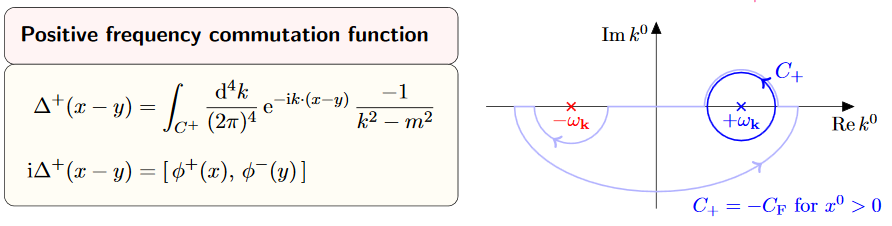graphics grabbed from Kocic 16

###### Proof

We compute as follows:

\begin{aligned} -i(2\pi)^{-(p+1)} \int \underset{C_+(\vec k)}{\oint} \frac{e^{ - i k_\mu (x-y)^\mu}}{ -k_\mu k^\mu - \left( \tfrac{m c}{\hbar} \right)^2 } d k_0 d^{p} k & = -i(2\pi)^{-(p+1)} \int \oint_{C_+(\vec k)} \frac{ e^{ -i k_0 x^0} e^{i \vec k \cdot (\vec x - \vec y)} }{ k_0^2 - \omega(\vec k)^2/c^2 } d k_0 d^p \vec k \\ & = -i(2\pi)^{-(p+1)} \int \underset{C_+(\vec k)}{\oint} \frac{ e^{ - i k_0 (x^0-y^0)} e^{i \vec k \cdot (\vec x - \vec y)} }{ ( k_0 - \omega_\epsilon(\vec k) ) ( k_0 + \omega_\epsilon(\vec k) ) } d k_0 d^p \vec k \\ & = (2\pi)^{-p} \int \frac{1}{2 \omega(\vec k)} e^{-i \omega(\vec k) (x^0-y^0)/c} e^{i \vec k \cdot (\vec x - \vec y)} d^p \vec k \,. \end{aligned}

The last step is application of Cauchy's integral formula, which says that the contour integral picks up the residue of the pole of the integrand at $+ \omega(\vec k)/c \in \mathbb{R} \subset \mathbb{C}$. The last line is $\Delta_H(x,y)$, by definition .

###### Proposition

(skew-symmetric part of Wightman propagator is the causal propagator)

The Wightman propagator for the Klein-Gordon equation on Minkowski spacetime (def. ) is of the form

(14)\begin{aligned} \Delta_H & = \tfrac{i}{2} \Delta_S + H \\ & = \tfrac{i}{2} \left( \Delta_+ - \Delta_- \right) + H \end{aligned} \,,

where

1. $\Delta_S$ is the causal propagator (prop. ), which is real (11) and skew-symmetric (prop. )

$(\Delta_S(x,y))^\ast = \Delta_S(x,y) \phantom{AA} \,, \phantom{AA} \Delta_S(y,x) = - \Delta_S(x,y)$
2. $H$ is real and symmetric

(15)$(H(x,y))^\ast = H(x,y) \phantom{AA} \,, \phantom{AA} H(y,x) = H(x,y)$
###### Proof

By applying Euler's formula to (12) we obtain

(16)\begin{aligned} \Delta_H(x,y) & = \frac{1}{(2\pi)^p} \int \frac{1}{2 \omega(\vec k)/c} e^{-i \omega(\vec k)(x^0 - y^0)/c + i \vec k \cdot (\vec x - \vec y) } \, d^p \vec k \\ & = \tfrac{i}{2} \underset{= \Delta_S(x,y)}{ \underbrace{ \frac{-1}{(2\pi)^p} \int \frac{1}{\omega(\vec k)/c} \sin\left( \omega(\vec k)(x^0 - y^0)/c \right) e^{i \vec k \cdot (\vec x - \vec y) } \, d^p \vec k }} \;+\; \underset{ \coloneqq H(x,y) }{ \underbrace{ \frac{1}{(2\pi)^p} \int \frac{1}{2 \omega(\vec k)/c} \cos\left( \omega(\vec k)(x^0 - y^0)/c \right) e^{i \vec k \cdot (\vec x - \vec y) } \, d^p \vec k }} \end{aligned}

On the left this identifies the causal propagator by (10), prop. .

The second summand changes, both under complex conjugation as well as under $(x-y) \mapsto (y-x)$, via change of integration variables $\vec k \mapsto - \vec k$ (because the cosine is an even function). This does not change the integral, and hence $H$ is symmetric.

$\,$

Feynman propagator

We have seen that the positive frequency component of the causal propagator $\Delta_S$ for the Klein-Gordon equation on Minkowski spacetime (prop. ) is the Wightman propagator $\Delta_H$ (def. ) given, according to prop. , by (14)

\begin{aligned} \Delta_H & = \tfrac{i}{2} \Delta_S + H \\ & = \tfrac{i}{2} \left( \Delta_+ - \Delta_- \right) + H \end{aligned} \,.

There is an evident variant of this combination, which will be of interest:

###### Definition

(Feynman propagator for Klein-Gordon equation on Minkowski spacetime)

$\Delta_F \coloneqq \tfrac{i}{2} \left( \Delta_+ + \Delta_- \right) + H$

where the first term is proportional to the sum of the advanced and retarded propagators (prop. ) and the second is the symmetric part of the Wightman propagator according to prop. .

Similarly the anti-Feynman propagator is

$\Delta_{\overline{F}} \coloneqq \tfrac{i}{2} \left( \Delta_+ + \Delta_- \right) - H \,.$

It follows immediately that:

###### Proposition

(Feynman propagator is symmetric)

The Feynman propagator $\Delta_F$ (def. ) is symmetric:

$\Delta_F(x,y) = \Delta_F(y,x) \,.$
###### Proof

By equation (9) in cor. we have that $\Delta_+ + \Delta_-$ is symmetric, and (15) in prop. says that $H$ is symmetric.

###### Proposition

(mode expansion for Feynman propagator of Klein-Gordon equation on Minkowski spacetime)

The Feynman propagator (def. ) for the Klein-Gordon equation on Minkowski spacetime is given by the following equivalent expressions

\begin{aligned} \Delta_F(x,y) & = \left\{ \array{ \frac{1}{(2\pi)^p} \int \frac{1}{\omega(\vec k)c} e^{-i\omega(\vec k)(x^0 - y^0)/c} e^{i \vec k \cdot (\vec x - \vec y)} \, d^p \vec k &\vert& (x^0 - y^0) \gt 0 \\ \frac{1}{(2\pi)^p} \int \frac{1}{2\omega(\vec k)c} e^{+i\omega(\vec k)(x^0 - y^0)/c} e^{i \vec k \cdot (\vec x - \vec y)} \, d^p \vec k &\vert& (x^0 - y^0) \lt 0 } \right. \\ & = \left\{ \array{ \Delta_H(x,y) &\vert& (x^0 - y^0) \gt 0 \\ \Delta_H(y,x) &\vert& (x^0 - y^0) \lt 0 } \right. \end{aligned}

Similarly the anti-Feynman propagator is equivalently given by

\begin{aligned} \Delta_{\overline{F}}(x,y) & = \left\{ \array{ \frac{-}{(2\pi)^p} \int \frac{1}{\omega(\vec k)c} e^{+i\omega(\vec k)(x^0 - y^0)/c} e^{i \vec k \cdot (\vec x - \vec y)} \, d^p \vec k &\vert& (x^0 - y^0) \gt 0 \\ \frac{-}{(2\pi)^p} \int \frac{1}{2\omega(\vec k)c} e^{-i\omega(\vec k)(x^0 - y^0)/c} e^{i \vec k \cdot (\vec x - \vec y)} \, d^p \vec k &\vert& (x^0 - y^0) \lt 0 } \right. \\ & = \left\{ \array{ -\Delta_H(y,x) &\vert& (x^0 - y^0) \gt 0 \\ -\Delta_H(x,y) &\vert& (x^0 - y^0) \lt 0 } \right. \end{aligned}
###### Proof

By the mode expansion of $\Delta_{\pm}$ from (2) and the mode expansion of $H$ from (16) we have

\begin{aligned} \Delta_F(x,y) & = \left\{ \array{ \underset{ = \tfrac{i}{2} \Delta_+(x,y) + 0 \;\text{for}\; (x^0 - y^0) \gt 0 }{ \underbrace{ \frac{- i}{(2\pi)^{p}} \int \frac{1}{2 \omega(\vec k)/c} \sin\left( \omega(\vec k)(x^0 - y^0)/c \right) e^{i \vec k \cdot (\vec x - \vec y) } \, d^p \vec k } } + \underset{ = H(x,y) }{ \underbrace{ \frac{1}{(2\pi)^p} \int \frac{1}{2 \omega(\vec k)/c} \cos\left( \omega(\vec k)(x^0 - y^0)/c \right) e^{i \vec k \cdot (\vec x - \vec y) } \, d^p \vec k } } &\vert& (x^0 - y^0) \gt 0 \\ \underset{ = 0 + \tfrac{i}{2}\Delta_-(x,y) \;\text{for}\; (x^0 - y^0) \lt 0 }{ \underbrace{ \frac{+ i}{(2\pi)^{p}} \int \frac{1}{2 \omega(\vec k)/c} \sin\left( \omega(\vec k)(x^0 - y^0)/c \right) e^{i \vec k \cdot (\vec x - \vec y) } \, d^p \vec k } } + \underset{ = H(x,y) }{ \underbrace{ \frac{1}{(2\pi)^p} \int \frac{1}{2 \omega(\vec k)/c} \cos\left( \omega(\vec k)(x^0 - y^0)/c \right) e^{i \vec k \cdot (\vec x - \vec y) } \, d^p \vec k } } &\vert& (x^0 - y^0) \lt 0 } \right. \\ & = \left\{ \array{ \frac{1}{(2\pi)^p} \int \frac{1}{2\omega(\vec k)c} e^{-i\omega(\vec k)(x^0 - y^0)/c} e^{i \vec k \cdot (\vec x - \vec y)} \, d^p \vec k &\vert& (x^0 - y^0) \gt 0 \\ \frac{1}{(2\pi)^p} \int \frac{1}{2\omega(\vec k)c} e^{+i\omega(\vec k)(x^0 - y^0)/c} e^{i \vec k \cdot (\vec x - \vec y)} \, d^p \vec k &\vert& (x^0 - y^0) \lt 0 } \right. \\ & = \left\{ \array{ \Delta_H(x,y) &\vert& (x^0 - y^0) \gt 0 \\ \Delta_H(y,x) &\vert& (x^0 - y^0) \lt 0 } \right. \end{aligned}

where in the second line we used Euler's formula. The last line follows by comparison with (12) and using that the integral over $\vec k$ is invariant under $\vec k \mapsto - \vec k$.

The computation for $\Delta_{\overline{F}}$ is the same, only now with a minus sign in front of the cosine:

\begin{aligned} \Delta_{\overline{F}}(x,y) & = \left\{ \array{ \underset{ = \tfrac{i}{2} \Delta_+(x,y) + 0 \;\text{for}\; (x^0 - y^0) \gt 0 }{ \underbrace{ \frac{- i}{(2\pi)^{p}} \int \frac{1}{2 \omega(\vec k)/c} \sin\left( \omega(\vec k)(x^0 - y^0)/c \right) e^{i \vec k \cdot (\vec x - \vec y) } \, d^p \vec k } } - \underset{ = H(x,y) }{ \underbrace{ \frac{1}{(2\pi)^p} \int \frac{1}{2 \omega(\vec k)/c} \cos\left( \omega(\vec k)(x^0 - y^0)/c \right) e^{i \vec k \cdot (\vec x - \vec y) } \, d^p \vec k } } &\vert& (x^0 - y^0) \gt 0 \\ \underset{ = 0 + \tfrac{i}{2}\Delta_-(x,y) \;\text{for}\; (x^0 - y^0) \lt 0 }{ \underbrace{ \frac{+ i}{(2\pi)^{p}} \int \frac{1}{2 \omega(\vec k)/c} \sin\left( \omega(\vec k)(x^0 - y^0)/c \right) e^{i \vec k \cdot (\vec x - \vec y) } \, d^p \vec k } } - \underset{ = H(x,y) }{ \underbrace{ \frac{1}{(2\pi)^p} \int \frac{1}{2 \omega(\vec k)/c} \cos\left( \omega(\vec k)(x^0 - y^0)/c \right) e^{i \vec k \cdot (\vec x - \vec y) } \, d^p \vec k } } &\vert& (x^0 - y^0) \lt 0 } \right. \\ & = \left\{ \array{ \frac{-1}{(2\pi)^p} \int \frac{1}{2\omega(\vec k)c} e^{+i\omega(\vec k)(x^0 - y^0)/c} e^{i \vec k \cdot (\vec x - \vec y)} \, d^p \vec k &\vert& (x^0 - y^0) \gt 0 \\ \frac{-1}{(2\pi)^p} \int \frac{1}{2\omega(\vec k)c} e^{-1i\omega(\vec k)(x^0 - y^0)/c} e^{i \vec k \cdot (\vec x - \vec y)} \, d^p \vec k &\vert& (x^0 - y^0) \lt 0 } \right. \\ & = \left\{ \array{ - \Delta_H(y,x) &\vert& (x^0 - y^0) \gt 0 \\ - \Delta_H(x,y) &\vert& (x^0 - y^0) \lt 0 } \right. \end{aligned}

As before for the causal propagator, there are equivalent reformulations of the Feynman propagator, which are useful for computations:

###### Proposition

(Feynman propagator as a Cauchy principal value)

The Feynman propagator and anti-Feynman propagator (def. ) for the Klein-Gordon equation on Minkowski spacetime is equivalently given by the following expressions, respectively:

\begin{aligned} \left. \array{ \Delta_F(x,y) \\ \Delta_{\overline{F}}(x,y) } \right\} & = \underset{ {\epsilon \in (0,\infty)} \atop {\epsilon \to 0} }{\lim} \frac{+i}{(2\pi)^{p+1}} \int \int_{-\infty}^\infty \frac{ e^{i k_\mu (x^\mu - y^\mu)} }{ - k_\mu k^\mu - \left( \tfrac{m c}{\hbar} \right)^2 \pm i \epsilon } \, d k_0 \, d^p \vec k \end{aligned}

where we have a limit of distributions as for the Cauchy principal value (this prop).

###### Proof

We compute as follows:

\begin{aligned} \underset{ {\epsilon \in (0,\infty)} \atop {\epsilon \to 0} }{\lim} \frac{i}{(2\pi)^{p+1}} \int \int_{-\infty}^\infty \frac{ e^{i k_\mu (x^\mu - y^\mu)} }{ - k_\mu k^\mu - \left( \tfrac{m c}{\hbar} \right)^2 \pm i \epsilon } \, d k_0 \, d^p \vec k & = \underset{ {\epsilon \in (0,\infty)} \atop {\epsilon \to 0} }{\lim} \frac{i}{(2\pi)^{p+1}} \int \int_{-\infty}^\infty \frac{ e^{i k_\mu (x^\mu - y^\mu)} }{ (k_0)^2 - \underset{ \coloneqq \omega_{\pm\epsilon}(\vec k)^2/c^2 }{\underbrace{ \left( \omega(\vec k)^2/c^2 \pm i \epsilon \right) }} } \, d k_0 \, d^p \vec k \\ & = \underset{ {\epsilon \in (0,\infty)} \atop {\epsilon \to 0} }{\lim} \frac{i}{(2\pi)^{p+1}} \int \int_{-\infty}^\infty \frac{ e^{i k_\mu (x^\mu - y^\mu)} }{ \left( k_0 - \omega_{\pm \epsilon}(\vec k)/c \right) \left( k_0 + \omega_{\pm \epsilon}(\vec k)/c \right) } \, d k_0 \, d^p \vec k \\ & = \left\{ \array{ \frac{\mp 1}{(2\pi)^p} \int \frac{1}{2\omega(\vec k)c} e^{\pm i\omega(\vec k)(x^0 - y^0)/c} e^{i \vec k \cdot (\vec x - \vec y)} \, d^p \vec k &\vert& (x^0 - y^0) \gt 0 \\ \frac{\mp 1}{(2\pi)^p} \int \frac{1}{2\omega(\vec k)c} e^{\mp i\omega(\vec k)(x^0 - y^0)/c} e^{i \vec k \cdot (\vec x - \vec y)} \, d^p \vec k &\vert& (x^0 - y^0) \lt 0 } \right. \\ & = \left\{ \array{ \Delta_F(x,y) \\ \Delta_{\overline{F}}(x,y) } \right. \end{aligned}

Here

1. In the first step we introduced the complex square root $\omega_{\pm \epsilon}(\vec k)$. For this to be compatible with the choice of non-negative square root for $\epsilon = 0$ in (1) we need to choose that complex square root whose complex phase is one half that of $\omega(\vec k)^2 - i \epsilon$ (instead of that plus π). This means that $\omega_{+ \epsilon}(\vec k)$ is in the upper half plane and $\omega_-(\vec k)$ is in the lower half plane.

2. In the third step we observe that

1. for $(x^0 - y^0) \gt 0$ the integrand decays for positive imaginary part and hence the integration over $k_0$ may be deformed to a contour which encircles the pole in the upper half plane;

2. for $(x^0 - y^0) \lt 0$ the integrand decays for negative imaginary part and hence the integration over $k_0$ may be deformed to a contour which encircles the pole in the lower half plane

and then apply Cauchy's integral formula which picks out $2\pi i$ times the residue a these poles.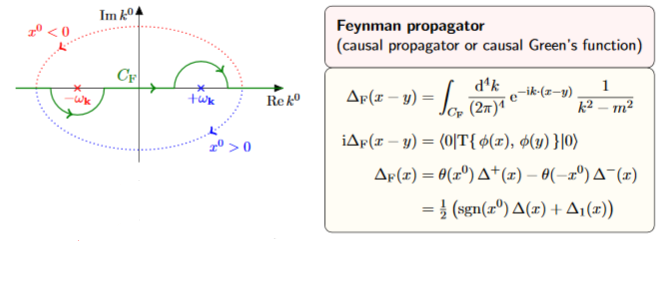Notice that when completing to a contour in the lower half plane we pick up a minus signs from the fact that now the contour runs clockwise.

3. In the fourth step we used prop. .

$\,$

$\,$

singular support and wave front sets

We now discuss the singular support and the wave front sets of the various propagators for the Klein-Gordon equation on Minkowski spacetime.

###### Proposition

(singular support of the causal propagator of the Klein-Gordon equation on Minkowski spacetime is the light cone)

The singular support of the causal propagator $\Delta_S$ for the Klein-Gordon equation on Minkowski spacetime, regarded via translation invariance as a generalized function in a single variable (?) is the light cone of the origin:

$supp_{sing}(\Delta_S) \;=\; \left\{ x \in \mathbb{R}^{p,1} \,\vert\, {\vert x\vert}^2_\eta = 0 \right\} \,.$
###### Proof

The statement follows immediately from the result (Gel’fand-Shilov 66, III 2.11 (7), p 294), see this prop.

We make this fully explicit now in the special case of spacetime dimension

$p + 1 = 3 + 1$

by computing an explicit form for the causal propagator in terms of the delta distribution, the Heaviside distribution and smooth Bessel functions.

Consider the formula for the causal propagator in terms of the mode expansion (10). Since the integrand here depends on the wave vector $\vec k$ only via its norm ${\vert \vec k\vert}$ and the angle $\theta$ it makes with the given spacetime vector via

$\vec k \cdot (\vec x - \vec y) \;=\; {\vert \vec k\vert} \, {\vert \vec x\vert} \, \cos(\theta)$

we may express the integration in terms of polar coordinates as follws:

\begin{aligned} \Delta_S(x - y) & = \frac{-1}{(2\pi)^p} \int \frac{1}{2 \omega(\vec k)/c} \sin\left( \omega(\vec k)(x^0 - y^0)/c \right) e^{i \vec k \cdot (\vec x - \vec y)} \, d^p \vec k \\ & = \frac{- vol_{S^{p-2}}}{(2\pi)^p} \underset{{\vert \vec k\vert} \in \mathbb{R}_{\geq 0}}{\int} \underset{ \theta \in [0,\pi] }{\int} \frac{ 1 }{ \omega(\vec k)/c } \sin\left( \omega(\vec k) (x^0 - y^0) /c \right) e^{ i {\vert \vec k\vert} {\vert \vec x - \vec y\vert} \cos(\theta) } {\vert \vec k\vert} ({\vert \vec k\vert} \sin(\theta))^{p-2} \, d \theta \wedge d {\vert \vec k\vert} \end{aligned}

In the special case of spacetime dimension $p + 1 = 3 + 1$ this becomes

(17)\begin{aligned} \Delta_S(x - y) & = \frac{- 2\pi}{(2\pi)^{3}} \underset{{\vert \vec k\vert} \in \mathbb{R}_{\geq 0}}{\int} \frac{ {\vert \vec k \vert}^2 }{ \omega(\vec k)/c } \sin\left( \omega(\vec k) (x^0 - y^0) /c \right) \underset{ = \tfrac{1}{i {\vert \vec k\vert}\, {\vert \vec x - \vec y\vert} } \left( e^{i {\vert \vec k\vert}\, {\vert \vec x - \vec y\vert}} - e^{-i {\vert \vec k\vert}\, {\vert \vec x - \vec y\vert}} \right) }{ \underbrace{ \underset{ \cos(\theta) \in [-1,1] }{\int} e^{ i {\vert \vec k\vert} {\vert \vec x - \vec y\vert} \cos(\theta) } d \cos(\theta) } } \wedge d {\vert \vec k \vert} \\ & = \frac{- 2}{(2\pi)^{2} {\vert \vec x - \vec y\vert}} \underset{{\vert \vec k\vert} \in \mathbb{R}_{\geq 0}}{\int} \frac{ {\vert \vec k \vert} }{ \omega(\vec k)/c } \sin\left( \omega(\vec k) (x^0 - y^0) /c \right) \sin\left( {\vert \vec k\vert}\, {\vert \vec x - \vec y\vert} \right) \, d {\vert \vec k\vert} \\ & = \frac{- 2}{(2\pi)^{2} {\vert \vec x - \vec y\vert}} \frac{d}{d {\vert \vec x - \vec y \vert } } \underset{{\vert \vec k\vert} \in \mathbb{R}_{\geq 0}}{\int} \frac{ 1 }{ \omega(\vec k)/c } \sin\left( \omega(\vec k) (x^0 - y^0) /c \right) \cos\left( {\vert \vec k\vert}\, {\vert \vec x - \vec y\vert} \right) \, d {\vert \vec k\vert} \\ & = \frac{- 1}{(2\pi)^{2} {\vert \vec x - \vec y\vert}} \frac{d}{d {\vert \vec x - \vec y \vert } } \underset{ \kappa \in \mathbb{R} }{\int} \frac{ 1 }{ \omega(\kappa)/c } \sin\left( \omega(\kappa) (x^0 - y^0) /c \right) \cos\left( \kappa\, {\vert \vec x - \vec y\vert} \right) \, d \kappa \\ & = \frac{- 1}{2(2\pi)^{2} {\vert \vec x - \vec y\vert}} \frac{d}{d {\vert \vec x - \vec y} \vert } \left( \underset{\coloneqq I_+}{ \underbrace{ \underset{ \kappa \in \mathbb{R} }{\int} \frac{ 1 }{ \omega(\kappa)/c } \sin\left( \omega(\kappa) (x^0 - y^0) /c + \kappa\, {\vert \vec x - \vec y\vert} \right) d\kappa } } + \underset{ \coloneqq I_- }{ \underbrace{ \underset{ \kappa \in \mathbb{R} }{\int} \frac{ 1 }{ \omega(\kappa)/c } \sin\left( \omega(\kappa) (x^0 - y^0) /c - \kappa\, {\vert \vec x - \vec y\vert} \right) \, d \kappa } } \right) \,. \end{aligned}

Here in the second but last step we renamed $\kappa \coloneqq {\vert \vec k\vert}$ and doubled the integration domain for convenience, and in the last step we used the trigonometric identity $\sin(\alpha) \cos(\beta)\;=\; \tfrac{1}{2} \left( \sin(\alpha + \beta) + \sin(\alpha - \beta) \right)$.

In order to further evaluate this, we parameterize the remaining components $(\omega/c, \kappa)$ of the wave vector by the dual rapidity $z$, via

$\left(\cosh(z)\right)^2 - \left( \sinh(z)\right)^2 = 1$

as

$\omega(\kappa)/c \;=\; \left( \tfrac{m c}{\hbar} \right) \cosh(z) \phantom{AA} \,, \phantom{AA} \kappa \;=\; \left( \tfrac{m c}{\hbar} \right) \sinh(z) \,,$

which makes use of the fact that $\omega(\kappa)$ is non-negative, by construction. This change of integration variables makes the integrals under the braces above become

(18)$I_\pm \;=\; \int_{-\infty}^\infty \sin\left( \tfrac{m c}{\hbar} \left( (x^0 - y^0) \cosh(z) \pm {\vert \vec x - \vec y\vert} \sinh(z) \right) \right) \, d z \,.$

Next we similarly parameterize the vector $x-y$ by its rapidity $\tau$. That parameterization depends on whether $x-y$ is spacelike or not, and if not, whether it is future or past directed.

First, if $x-y$ is spacelike in that ${\vert x-y\vert}^2_\eta \gt 0$ then we may parameterize as

$(x^0 - y^0) = \sqrt{{\vert x-y\vert}^2_\eta} \sinh(\tau) \phantom{AA} \,, \phantom{AA} {\vert \vec x - \vec y\vert} = \sqrt{ {\vert x-y\vert}^2_\eta} \cosh(\tau)$

which yields

\begin{aligned} I_{\pm} & = \int_{-\infty}^\infty \sin\left( \tfrac{m c}{\hbar} \sqrt{ {\vert x-y\vert}^2_\eta } \left( \sinh(\tau) \cosh(z) \pm \cosh(\tau) \sinh(z) \right) \right) \, d z \\ & = \int_{-\infty}^\infty \sin\left( \tfrac{m c}{\hbar} \sqrt{ {\vert x-y\vert}^2_\eta} \left( \sinh\left( \tau \pm z\right) \right) \right) \, d z \\ & = \int_{-\infty}^\infty \sin\left( \tfrac{m c}{\hbar} \sqrt{ {\vert x-y\vert}^2_\eta } \left( \sinh\left( z \right) \right) \right) \, d z \\ & = 0 \,, \end{aligned}

where in the last line we observe that the integrand is a skew-symmetric function of $z$.

Second, if $x-y$ is timelike with $(x^0 - y^0) \gt 0$ then we may parameterize as

$(x^0 - y^0) = \sqrt{ -{\vert x-y\vert}^2_\eta} \cosh(\tau) \phantom{AA} \,, \phantom{AA} {\vert \vec x - \vec y\vert} = \sqrt{ -{\vert x - y\vert}^2_\eta } \sinh(\tau)$

which yields

(19)\begin{aligned} I_\pm & = \int_{-\infty}^\infty \sin\left( \tfrac{m c}{\hbar} \left( (x^0 - y^0) \cosh(z) \pm {\vert \vec x - \vec y\vert} \sinh(z) \right) \right) \, d z \\ & = \int_{-\infty}^\infty \sin\left( \sqrt{ - {\vert x-y\vert}^2_\eta } \tfrac{m c}{\hbar} \left( \cosh(\tau)\cosh(z) \pm \cosh(\tau) \sinh(z) \right) \right) \, d z \\ & = \int_{-\infty}^\infty \sin\left( \sqrt{ - {\vert x-y\vert}^2_\eta } \tfrac{m c}{\hbar} \left( \cosh(z \pm \tau) \right) \right) \, d z \\ & = \pi J_0\left( \sqrt{ - {\vert x-y\vert}^2_\eta} \tfrac{m c}{\hbar} \right) \end{aligned} \,.

Here in the last line we identified the integral representation of the Bessel function $J_0$ of order 0 (see here). The important point here is that this is a smooth function.

Similarly, if $x-y$ is timelike with $(x^0 - y^0) \lt 0$ then the same argument yields

$I_\pm = - \pi J_0\left( \sqrt{ - {\vert x-y\vert}^2_\eta} \tfrac{m c}{\hbar} \right)$

In conclusion, the general form of $I_\pm$ is

$I_\pm = \pi sgn(x^0 - y^0) \Theta\left( -{\vert x-y\vert}^2_\eta \right) J_0\left( \sqrt{ - {\vert x-y\vert}^2_\eta } \tfrac{m c}{\hbar} \right) \,.$

Therefore we end up with

(20)\begin{aligned} \Delta_S(x,y) & = \frac{1}{4 \pi {\vert \vec x - \vec y\vert}} \frac{d}{d {\vert \vec x - \vec y\vert}} sgn(x^0) \Theta\left( -{\vert x-y\vert}^2_\eta \right) J_0\left( \sqrt{ -{\vert x-y\vert}^2_\eta } \tfrac{m c}{\hbar} \right) \\ & = \frac{-1}{2 \pi } \frac{d}{d (-{\vert x-y\vert}^2_\eta)} sgn(x^0) \Theta\left( -{\vert x-y\vert}^2_\eta \right) J_0\left( \sqrt{-{\vert x-y \vert}^2_\eta} \tfrac{m c}{\hbar} \right) \\ & = -\frac{1}{2 \pi } \frac{d}{d (- \vert x-y\vert^2_{\eta})} sgn(x^0) \Theta\left( - {\vert x - y\vert}^2_\eta \right) J_0\left( \tfrac{m c}{\hbar} \sqrt{ -{\vert x-y\vert}^2_\eta } \right) \\ & = \frac{-1}{2\pi} sgn(x^0) \left( \delta\left( -{\vert x-y\vert}^2_\eta \right) \;-\; \Theta\left( -{\vert x-y\vert}^2_\eta \right) \frac{d}{d \left({-\vert x-y\vert}^2_\eta\right) } J_0\left( \tfrac{m c}{\hbar} \sqrt{ -{\vert x-y\vert}^2_\eta } \right) \right) \end{aligned}
###### Proposition

(singular support of the Wightman propagator of the Klein-Gordon equation on Minkowski spacetime is the light cone)

The singular support of the Wightman propagator $\Delta_H$ (def. ) for the Klein-Gordon equation on Minkowski spacetime, regarded via translation invariance as a distribution in a single variable, is the light cone of the origin:

$supp_{sing}(\Delta_H) = \left\{ x \in \mathbb{R}^{p,1} \;\vert\; {\vert x\vert}^2_\eta = 0 \right\} \,.$
###### Proof

The statement follows immediately from the result (Gel’fand-Shilov 66, III 2.11 (7), p 294), see this prop..

We make this fully explicit now in the special case of spacetime dimension

$p + 1 = 3 + 1$

by computing an explicit form for the causal propagator in terms of the delta distribution, the Heaviside distribution and smooth Bessel functions.

By (16) we have

\begin{aligned} \Delta_H(x,y) & = \tfrac{i}{2} \underset{= \Delta_S(x,y)}{ \underbrace{ \frac{-1}{(2\pi)^p} \int \frac{1}{\omega(\vec k)/c} \sin\left( \omega(\vec k)(x^0 - y^0)/c \right) e^{i \vec k \cdot (\vec x - \vec y) } \, d^p \vec k }} \;+\; \underset{ \coloneqq H(x,y) }{ \underbrace{ \frac{1}{(2\pi)^p} \int \frac{1}{2 \omega(\vec k)/c} \cos\left( \omega(\vec k)(x^0 - y^0)/c \right) e^{i \vec k \cdot (\vec x - \vec y) } \, d^p \vec k }} \end{aligned}

The first summand, proportional to the causal propagator, which we computed as (20) in prop. to be

$\tfrac{i}{2}\Delta_S(x,y) \;=\; \frac{-i}{4\pi} sgn(x^0) \left( \delta\left( -{\vert x-y\vert}^2_\eta \right) \;-\; \Theta\left( -{\vert x-y\vert}^2_\eta \right) \frac{d}{d \left({-\vert x-y\vert}^2_\eta\right) } J_0\left( \tfrac{m c}{\hbar} \sqrt{ -{\vert x-y\vert}^2_\eta } \right) \right) \,.$

The second term is computed in a directly analogous fashion: The integrals $I_\pm$ from (18) are now

$I_\pm \coloneqq \int_{-\infty}^\infty \cos\left( \tfrac{m c}{\hbar} \left( (x^0 - y^0) \cosh(z) \pm {\vert \vec x - \vec y\vert} \sinh(z) \right) \right) \, d z$

Parameterizing by rapidity, as in the proof of prop. , one finds that for timelike $x-y$ this is

\begin{aligned} I_\pm & = \int_{-\infty}^\infty \cos\left( \tfrac{m c}{\hbar} \sqrt{ {\vert x-y\vert}^2_\eta } \left( \cosh\left( z \right) \right) \right) \, d z \\ & = - \pi N_0 \left( \tfrac{m c}{\hbar} \sqrt{ {\vert x-y\vert}^2_\eta } \right) \end{aligned}

while for spacelike $x-y$ it is

\begin{aligned} I_\pm & = \int_{-\infty}^\infty \cos\left( \tfrac{m c}{\hbar} \sqrt{ {\vert x-y\vert}^2_\eta } \left( \sinh\left( z \right) \right) \right) \, d z \\ & = 2 K_0 \left( \tfrac{m c}{\hbar} \sqrt{ {\vert x-y\vert}^2_\eta } \right) \,, \end{aligned}

where we identified the integral representations of the Neumann function $N_0$ (see here) and of the modified Bessel function $K_0$ (see here).

As for the Bessel function $J_0$ in (19) the key point is that these are smooth functions. Hence we conclude that

$H(x,y) \;\propto\; \frac{d}{d \left( {\vert x-y\vert}^2_\eta \right)} \left( -\Theta\left( -{\vert x-y\vert}^2_\eta \right) N_0 \left( \tfrac{m c}{\hbar} \sqrt{ {\vert x-y\vert}^2_\eta } \right) + \Theta\left( {\vert x-y\vert}^2_\eta \right) \tfrac{2}{\pi} K_0 \left( \tfrac{m c}{\hbar} \sqrt{ {\vert x-y\vert}^2_\eta } \right) \right) \,.$

This expression has singularities on the light cone due to the step functions. In fact the expression being differentiated is continuous at the light cone (Scharf 95 (2.3.34)), so that the singularity on the light cone is not a delta distribution singularity from the derivative of the step functions. Accordingly it does not cancel the singularity of $\tfrac{i}{2}\Delta_S(x,y)$ as above, and hence the singular support of $\Delta_H$ is still the whole light cone.

###### Proposition

(singular support of Feynman propagator for Klein-Gordon equation on Minkowski spacetime)

The singular support of the Feynman propagator $\Delta_H$ and of the anti-Feynman propagator $\Delta_{\overline{F}}$ (def. ) for the Klein-Gordon equation on Minkowski spacetime, regarded via translation invariance as a distribution in a single variable, is the light cone of the origin:

$\left. \array{ supp_{sing}(\Delta_F) \\ supp_{sing}(\Delta_{\overline{F}}) } \right\} = \left\{ x \in \mathbb{R}^{p,1} \;\vert\; {\vert x\vert}^2_\eta = 0 \right\} \,.$
###### Proof

The statement follows immediately from the result (Gel’fand-Shilov 66, III 2.8 (8) and (9), p 289), see this prop..

###### Proposition

(wave front sets of propagators of Klein-Gordon equation on Minkowski spacetime)

The wave front set of the various propagators for the Klein-Gordon equation on Minkowski spacetime, regarded, via translation invariance, as distributions in a single variable, are as follows:

• the causal propagator $\Delta_S$ (prop. ) has wave front set all pairs $(x,k)$ with $x$ and $k$ both on the lightcone:
$WF(\Delta_S) = \left\{ (x,k) \,\vert\, {\vert x\vert}^2_\eta = 0 \;\text{and} \; {\vert k\vert}^2_\eta = 0 \; \text{and} \, k \neq 0 \right\}$-• the Wightman propagator $\Delta_H$ (def. ) has wave front set all pairs $(x,k)$ with $x$ and $k$ both on the light cone and $k^0 \gt 0$:
$WF(\Delta_H) = \left\{ (x,k) \,\vert\, {\vert x\vert}^2_\eta = 0 \;\text{and} \; {\vert k\vert}^2_\eta = 0 \; \text{and} \; k^0 \gt 0 \right\}$• the Feynman propagator $\Delta_S$ (def. ) has wave front set all pairs $(x,k)$ with $x$ and $k$ both on the light cone and $\pm k_0 \gt 0 \;\Leftrightarrow\; \pm x^0 \gt 0$
$WF(\Delta_H) = \left\{ (x,k) \,\vert\, {\vert x\vert}^2_\eta = 0 \;\text{and} \; {\vert k\vert}^2_\eta = 0 \; \text{and} \; \left( \pm k_0 \gt 0 \;\Leftrightarrow\; \pm x^0 \gt 0 \right) \right\}$###### Proof

First regarding the causal propagator:

By prop. the singular support of $\Delta_S$ is the light cone.

Since the causal propagator is a solution to the homogeneous Klein-Gordon equation, the propagation of singularities theorem says that also all wave vectors in the wave front set are lightlike. Hence it just remains to show that all non-vanishing lightlike wave vectors based on the lightcone in spacetime indeed do appear in the wave front set.

To that end, let $b \in C^\infty_{cp}(\mathbb{R}^{p,1})$ be a bump function whose compact support includes the origin.

For $a \in \mathbb{R}^{p,1}$ a point on the light cone, we need to determine the decay property of the Fourier transform of $x \mapsto b(x-a)\Delta_S(x)$. This is the convolution of distributions of $\hat b(k)e^{i k_\mu a^\mu}$ with $\widehat \Delta_S(k)$. By prop. we have

$\widehat \Delta_{S}(k) \;\propto\; \delta\left( -k_\mu k^\mu - \left( \tfrac{m c}{\hbar} \right)^2 \right) sgn(k_0) \,.$

This means that the convolution product is the smearing of the mass shell by $\widehat b(k)e^{i k_\u a^\mu}$.

Since the mass shell asymptotes to the light cone, and since $e^{i k_\mu a^\mu} = 1$ for $k$ on the light cone (given that $a$ is on the light cone), this implies the claim.

Now for the Wightman propagator:

By def. its Fourier transform is of the form

$\widehat \Delta_H(k) \;\propto\; \delta\left( k_\mu k^\mu + m^2 \right) \Theta( -k_0 )$

Moreover, its singular support is also the light cone (prop. ).

Therefore now same argument as before says that the wave front set consists of wave vectors $k$ on the light cone, but now due to the step function factor $\Theta(-k_0)$ it must satisfy $0 \leq - k_0 = k^0$.

Finally regarding the Feynman propagator:

by prop. the Feynman propagator coincides with the positive frequency Wightman propagator for $x^0 \gt 0$ and with the “negative frequency Hadamard operator” for $x^0 \lt 0$. Therefore the form of $WF(\Delta_F)$ now follows directly with that of $WF(\Delta_H)$ above.

### For Dirac operator on Minkowski spacetime

Finally we observe that the propagators for the Dirac field on Minkowski spacetime follow immediately from the propagators for the scalar field:

###### Proposition

(advanced and retarded propagator for Dirac equation on Minkowski spacetime)

Consider the Dirac operator on Minkowski spacetime, which in Feynman slash notation reads

\begin{aligned} D & \coloneqq -i {\partial\!\!\!/\,} + \tfrac{m c}{\hbar} \\ & = -i \gamma^\mu \frac{\partial}{\partial x^\mu} + \tfrac{m c}{\hbar} \end{aligned} \,.

Its advanced and retarded propagators (def. ) are the derivatives of distributions of the advanced and retarded propagators $\Delta_\pm$ for the Klein-Gordon equation (prop. ) by ${\partial\!\!\!/\,} + m$:

$\Delta_{D, \pm} \;=\; \left( -i{\partial\!\!\!/\,} - \tfrac{m c}{\hbar} \right) \Delta_{\pm} \,.$

Hence the same is true for the causal propagator:

$\Delta_{D, S} \;=\; \left( -i{\partial\!\!\!/\,} - \tfrac{m c}{\hbar} \right) \Delta_{S} \,.$
###### Proof

Applying a differential operator does not change the support of a smooth function, hence also not the support of a distribution. Therefore the uniqueness of the advanced and retarded propagators (prop. ) together with the translation-invariance and the anti-formally self-adjointness of the Dirac operator (as for the Klein-Gordon operator (?) implies that it is sufficent to check that applying the Dirac operator to the $\Delta_{D, \pm}$ yields the delta distribution. This follows since the Dirac operator squares to the Klein-Gordon operator:

\begin{aligned} \left( -i{\partial\!\!\!/\,} + \tfrac{m c}{\hbar} \right) \Delta_{D, \pm} & = \underset{ = \Box - \left(\tfrac{m c}{\hbar}\right)^2}{ \underbrace{ \left( -i{\partial\!\!\!/\,} + \tfrac{m c}{\hbar} \right) \left( -i{\partial\!\!\!/\,} - \tfrac{m c}{\hbar} \right) } } \Delta_{\pm} \\ & = \delta \end{aligned} \,.

Similarly we obtain the other propagators for the Dirac field from those of the real scalar field:

###### Definition

(Wightman propagator for Dirac operator on Minkowski spacetime)

The Wightman propagator for the Dirac operator on Minkowski spacetime is the positive frequency part of the causal propagator (prop. ), hence the derivative of distributions of the Wightman propagator for the Klein-Gordon field (def. ) by the Dirac operator:

\begin{aligned} \left( -i{\partial\!\!\!/\,} + \tfrac{m c}{\hbar} \right)\Delta_{H}(x,y) & = \frac{1}{(2\pi)^p} \int \delta\left( k_\mu k^\mu + m^2 \right) \Theta( -k_0 ) ( {k\!\!\!/\,} + \tfrac{m c}{\hbar}) e^{i k_\mu (x^\mu-y^\mu) } \, d^{p+1} k \\ & = \frac{1}{(2\pi)^p} \int \frac{ \gamma^0 \omega(\vec k)/c + \vec \gamma \cdot \vec k + \tfrac{m c}{\hbar} }{2 \omega(\vec k)/c} e^{-i \omega(\vec k)(x^0 - y^0)/c + i \vec k \cdot (\vec x - \vec y) } \, d^p \vec k \,. \end{aligned}

Here we used the expression (?) for the Wightman propagator of the Klein-Gordon equation.

###### Definition

(Feynman propagator for Dirac operator on Minkowski spacetime)

The Feynman propagator for the Dirac operator on Minkowski spacetime (also called the electron propagator) is the linear combination

$\Delta_{D, F} \;\coloneqq\; \Delta_{D,H} + i \Delta_{D, -}$

of the Wightman propagator (def. ) and the retarded propagator (prop. ). By prop. this means that it is the derivative of distributions of the Feynman propagator of the Klein-Gordon equation (def. ) by the Dirac operator

\begin{aligned} \Delta_{D, F} & = \left( -i{\partial\!\!\!/\,} + \tfrac{m c}{\hbar} \right)\Delta_{F}(x,y) & = \underset{ {\epsilon \in (0,\infty)} \atop {\epsilon \to 0} }{\lim} \frac{-i}{(2\pi)^{p+1}} \int \int_{-\infty}^\infty \frac{ \left( {k\!\!\!/\,} + \tfrac{m c}{\hbar} \right) e^{i k_\mu (x^\mu - y^\mu)} }{ - k_\mu k^\mu - \left( \tfrac{m c}{\hbar} \right)^2 + i \epsilon } \, d k_0 \, d^p \vec k \,. \end{aligned}

### In Feynman amplitudes

###### Example

(Feynman amplitudes in causal perturbation theory – example of QED)

In perturbative quantum field theory, Feynman diagrams are labeled multigraphs that encode products of Feynman propagators, called Feynman amplitudes (this prop.) which in turn contribute to probability amplitudes for physical scattering processes – scattering amplitudes:

The Feynman amplitudes are the summands in the Feynman perturbation series-expansion of the scattering matrix

$\mathcal{S} \left( S_{int} \right) = \underset{k \in \mathbb{N}}{\sum} \frac{1}{k!} \frac{1}{(i \hbar)^k} T( \underset{k \, \text{factors}}{\underbrace{S_{int}, \cdots , S_{int}}} )$

of a given interaction Lagrangian density $L_{int}$.

The Feynman amplitudes are the summands in an expansion of the time-ordered products $T(\cdots)$ of the interaction with itself, which, away from coincident vertices, is given by the star product of the Feynman propagator $\Delta_F$ (this prop.), via the exponential contraction

$T(S_{int}, S_{int}) \;=\; prod \circ \exp \left( \hbar \int \Delta_{F}^{a b}(x,y) \frac{\delta}{\delta \mathbf{\Phi}^a(x)} \otimes \frac{\delta}{\delta \mathbf{\Phi}(y)} \right) ( S_{int} \otimes S_{int} ) \,.$

Each edge in a Feynman diagram corresponds to a factor of a Feynman propagator in $T( \underset{k \, \text{factors}}{\underbrace{S_{int} \cdots S_{int}}} )$, being a distribution of two variables; and each vertex corresponds to a factor of the interaction Lagrangian density at $x_i$.

For example quantum electrodynamics in Gaussian-averaged Lorenz gauge involves (via this example):

1. the Dirac field modelling the electron, with Feynman propagator called the electron propagator (this def.), here to be denoted

$\Delta \phantom{AAAA} \text{electron propagator}$
2. the electromagnetic field modelling the photon, with Feynman propagator called the photon propagator (this prop.), here to be denoted

$G \phantom{AAAA} \text{photon propagator}$
3. $L_{int} \;=\; \underset{ \text{interaction} }{ \underbrace{ i g (\gamma^\mu)^\alpha{}_\beta } } \, \underset{ { \text{incoming} \atop \text{electron} } \atop \text{field} }{\underbrace{\overline{\psi_\alpha}}} \; \underset{ { \, \atop \text{photon} } \atop \text{field} }{\underbrace{a_\mu}} \; \underset{ {\text{outgoing} \atop \text{electron} } \atop \text{field} }{\underbrace{\psi^\beta}}$

The Feynman diagram for the electron-photon interaction alone is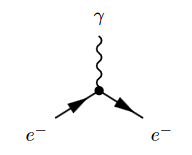where the solid lines correspond to the electron, and the wiggly line to the photon. The corresponding product of distributions is (written in generalized function-notation)

$\underset{ \text{loop order} }{ \underbrace{ \hbar^{3/2-1} } } \underset{ \text{electron-photon} \atop \text{interaction} }{ \underbrace{ i g (\gamma^\mu)^\alpha{}_\beta } } \,. \, \underset{ {\text{incoming} \atop \text{electron}} \atop \text{propagator} }{ \underbrace{ \overline{\Delta(-,x)}_{-, \alpha} } } \underset{ { \, \atop \text{photon} } \atop \text{propagator} }{ \underbrace{ G(x,-)_{\mu,-} } } \underset{ { \text{outgoing} \atop \text{electron} } \atop \text{propagator} }{ \underbrace{ \Delta(x,-)^{\beta, -} } }$

Hence a typical Feynman diagram in the QED Feynman perturbation series induced by this electron-photon interaction looks as follows: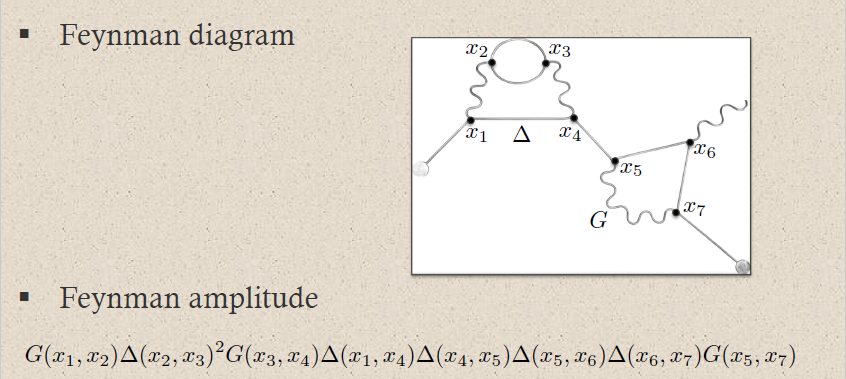where on the bottom the corresponding Feynman amplitude product of distributions is shown; now notationally suppressing the contraction of the internal indices and all prefactors.

For instance the two solid edges between the vertices $x_2$ and $x_3$ correspond to the two factors of $\Delta(x_2,x_2)$: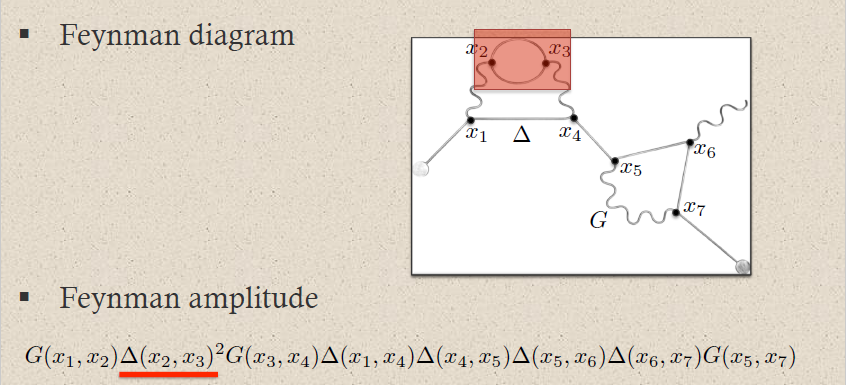This way each sub-graph encodes its corresponding subset of factors in the Feynman amplitude: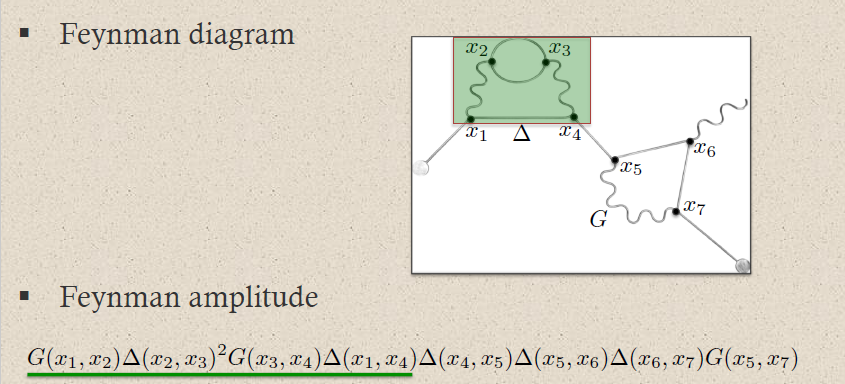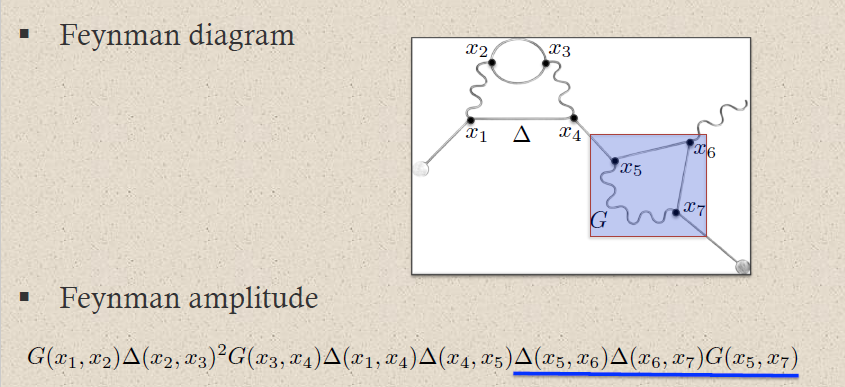graphics grabbed from Brouder 10

A priori this product of distributions is defined away from coincident vertices: $x_i \neq x_j$. The definition at coincident vertices $x_i = x_j$ requires a choice of extension of distributions to the diagonal locus. This choice is the ("re-")normalization of the Feynman amplitude.

### As a zeta function

needs harmonization

From another perspective, the loop contributions of Feynman diagrams are typically would-be traces over inverse powers $H^{-n}$ of the relativistic particle Hamiltonian.

For instance for the free scalar particle of mass $m$ in 4d Minkowski spacetime the 1-loop vacuum amplitude is the regularized trace over the Feynman propagator

$\propto \int d^4 \mathbf{p} \; \frac{1}{\mathbf{p}^2 - m^2}$

where the integral would naively be over all of $\mathbb{R}^4$, which is of course not well defined. The integrand here is typically called the Feynman propagator or propagator for short (e.g. Grozin 05, section 2.1 Kleinert 11, 8.1). See at Feynman diagram – For finitely many degrees of freedoms for how this comes about.

Several methods are considered for regularizing, hence making sense of it as a finite expression. One of these is zeta function regularization (also “analytic regularization/renormalization” Speer 71). Here one notices that the zeta function of the wave operator/Laplace operator $H = \mathbf{p}^2 + m^2$ is well-defined for $\Re(s) \gt 1$ by the naive trace

$\hat \zeta_H(s)\coloneqq Tr_{reg}( H^{-s} )$

and defined from there by analytic continuation on allmost all of the complex plane. The special value at $s = 1$ (or its principal value) is the regularized Feynman propagator. See (BCEMZ 03, section 2.4.2). For the example of the above basic Feynman propagator see e.g. Grozin 05, section 2.1

Representing the (completed) zeta function here are the Mellin transform of some theta function – which in the present case is the partition function $t\mapsto Tr_{reg} \exp(-t H)$ of the worldline formalism of the given theory, is what in the physics literature is known as the Schwinger parameter-formulation

$Tr_{reg} H^{-s} = \int_0^\infty t^{s-1} Tr\, \exp(-t H)\,d t \,.$
context/function field analogytheta function $\theta$zeta function $\zeta$ (= Mellin transform of $\theta(0,-)$)L-function $L_{\mathbf{z}}$ (= Mellin transform of $\theta(\mathbf{z},-)$)eta function $\eta$special values of L-functions
physics/2d CFTpartition function $\theta(\mathbf{z},\mathbf{\tau}) = Tr(\exp(-\mathbf{\tau} \cdot (D_\mathbf{z})^2))$ as function of complex structure $\mathbf{\tau}$ of worldsheet $\Sigma$ (hence polarization of phase space) and background gauge field/source $\mathbf{z}$analytically continued trace of Feynman propagator $\zeta(s) = Tr_{reg}\left(\frac{1}{(D_{0})^2}\right)^s = \int_{0}^\infty \tau^{s-1} \,\theta(0,\tau)\, d\tau$analytically continued trace of Feynman propagator in background gauge field $\mathbf{z}$: $L_{\mathbf{z}}(s) \coloneqq Tr_{reg}\left(\frac{1}{(D_{\mathbf{z}})^2}\right)^s = \int_{0}^\infty \tau^{s-1} \,\theta(\mathbf{z},\tau)\, d\tau$analytically continued trace of Dirac propagator in background gauge field $\mathbf{z}$ $\eta_{\mathbf{z}}(s) = Tr_{reg} \left(\frac{sgn(D_{\mathbf{z}})}{ { \vert D_{\mathbf{z}} } \vert }\right)^s$regularized 1-loop vacuum amplitude $pv\, L_{\mathbf{z}}(1) = Tr_{reg}\left(\frac{1}{(D_{\mathbf{z}})^2}\right)$ / regularized fermionic 1-loop vacuum amplitude $pv\, \eta_{\mathbf{z}}(1)= Tr_{reg} \left( \frac{D_{\mathbf{z}}}{(D_{\mathbf{z}})^2} \right)$ / vacuum energy $-\frac{1}{2}L_{\mathbf{z}}^\prime(0) = Z_H = \frac{1}{2}\ln\;det_{reg}(D_{\mathbf{z}}^2)$
Riemannian geometry (analysis)zeta function of an elliptic differential operatorzeta function of an elliptic differential operatoreta function of a self-adjoint operatorfunctional determinant, analytic torsion
complex analytic geometrysection $\theta(\mathbf{z},\mathbf{\tau})$ of line bundle over Jacobian variety $J(\Sigma_{\mathbf{\tau}})$ in terms of covering coordinates $\mathbf{z}$ on $\mathbb{C}^g \to J(\Sigma_{\mathbf{\tau}})$zeta function of a Riemann surfaceSelberg zeta functionDedekind eta function
arithmetic geometry for a function fieldGoss zeta function (for arithmetic curves) and Weil zeta function (in higher dimensional arithmetic geometry)
arithmetic geometry for a number fieldHecke theta function, automorphic formDedekind zeta function (being the Artin L-function $L_{\mathbf{z}}$ for $\mathbf{z} = 0$ the trivial Galois representation)Artin L-function $L_{\mathbf{z}}$ of a Galois representation $\mathbf{z}$, expressible “in coordinates” (by Artin reciprocity) as a finite-order Hecke L-function (for 1-dimensional representations) and generally (via Langlands correspondence) by an automorphic L-function (for higher dimensional reps)class number $\cdot$ regulator
arithmetic geometry for $\mathbb{Q}$Jacobi theta function ($\mathbf{z} = 0$)/ Dirichlet theta function ($\mathbf{z} = \chi$ a Dirichlet character)Riemann zeta function (being the Dirichlet L-function $L_{\mathbf{z}}$ for Dirichlet character $\mathbf{z} = 0$)Artin L-function of a Galois representation $\mathbf{z}$ , expressible “in coordinates” (via Artin reciprocity) as a Dirichlet L-function (for 1-dimensional Galois representations) and generally (via Langlands correspondence) as an automorphic L-function

Textbook accounts for quantum fields on Minkowski spacetime includes

An concise overview of the Green functions of the Klein-Gordon operator, hence of the Feynman propagator, advanced propagator, retarded propagator, causal propagator etc. is given in

• Mikica Kocic, Invariant Commutation and Propagation Functions Invariant Commutation and Propagation Functions, 2016 (pdf)

Discussion on general globally hyperbolic spacetimes is in

• Marek Radzikowski, Micro-local approach to the Hadamard condition in quantum field theory on curved space-time, Commun. Math. Phys. 179 (1996), 529–553 (Euclid)

where the issue with the underlying Wightman propagators was settled, and reviewed for instance in

• A. Bytsenko, G. Cognola, Emilio Elizalde, Valter Moretti, S. Zerbini, section 2 of Analytic Aspects of Quantum Fields, World Scientific Publishing, 2003, ISBN 981-238-364-6

Lecture notes (mostly for the case over Minkowski spacetime) include

• Andrey Grozin, Lectures on QED and QCD (arXiv:hep-ph/0508242)

• Green functions and propagators (pdf)

• Green functions for the Klein-Gordon operator (pdf)

• Hagen Kleinert, V. Schulte-Frohlinde, Critical properties of $\phi^4$-Theories 2001 (pdf)

An overview of the Green functions of the Klein-Gordon operator, hence of the Feynman propagator, advanced propagator, retarded propagator, causal propagator etc. is given in

• Mikica Kocic, Invariant Commutation and Propagation Functions Invariant Commutation and Propagation Functions, 2016 (pdf)

The zeta function regularization method originates around

• Eugene Speer, On the structure of Analytic Renormalization, Comm. math. Phys. 23, 23-36 (1971) (Euclid)

and a comprehensive discussion is in (BCEMZ 03, section 2).

Discussion of zeta functions of Dirac operators in 2d includes

• Michael McGuigan, Riemann Hypothesis and Short Distance Fermionic Green’s Functions (arXiv:math-ph/0504035)

Lecture notes concerning 1-loop vacuum amplitudes for the string include

• The IIA/B superstring one-loop vacuum amplitude (pdf)